# KMeans as a classifier for the WIFI and MNIST datasets – III – KMeans as a classifier for the WIFI-example

In the previous articles of this series

I applied KMeans to the „WIFI“ dataset – a small and rather simple training set of the UCI Irvine. The 7 dimensional feature space for this example is defined by the strength of seven different WLAN-signals. The data samples are labeled by numbers specifying four different rooms. We just have 2000 samples. But „simple“ does not mean that one cannot learn something of it.

An elbow and a silhouette analysis indicated that the data can well be described by 4 to 5 clusters.
A detailed PCA analysis helped us to understand that the data could basically be described by two primary components whose axes were defined by a diagonal in a two-dimensional sub-space of the original feature space (defined by the features „WLAN-0“ and „WLAN-3“) plus an orthogonal axis (defined by „WLAN-4“). As the data points segregated into well separated clusters in the coordinate system of the primary components we could expect that they also segregate well when projected onto two 2-dim sub-spaces of the feature space defined by the signal combinations {WLAN-0/WLAN-4} and {WLAN-3/WLAN-4}.

So we projected the results of a KMeans analysis into a 2-dim sub-space of the 7-dim feature space spanned by two selected „features“ (WLAN signals WLAN-0 and WLAN-4). And indeed: For 2 special signal- or feature-combinations we saw a field with 4 to 5 well separated clusters.

As we have well separated clusters – either in sub-planes of the feature space or the space defined by the dominant two PCA components – a legitimate question is: Can we use the cluster information for classifying?

# Clusters and classification

In the special example of the WIFI data the data points can obviously be divided into different „groups“ filled by data points which belong to a certain „label“ or class (in the WIFI case: a room):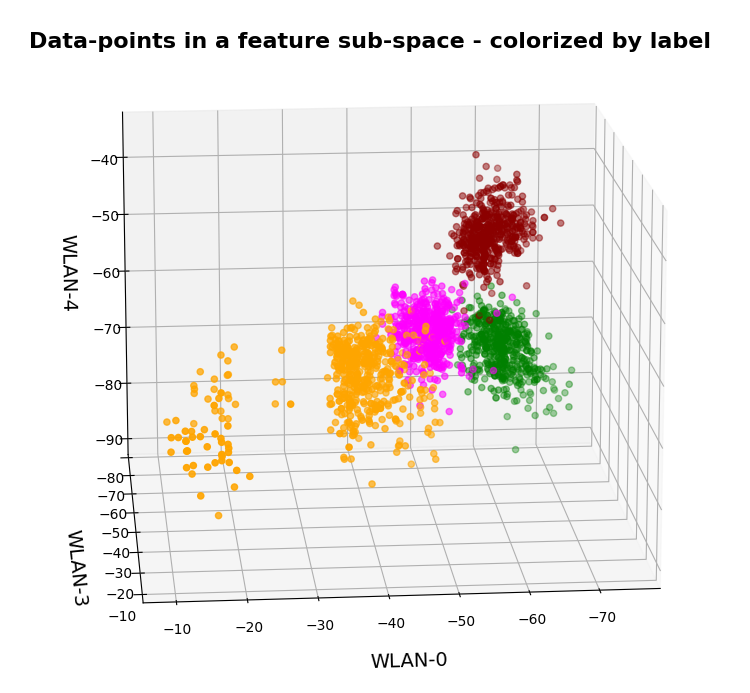In the plots above the colorization of the data points is given by their label in the plot above. However:
Does this mean that these spatially separated „groups“ coincide with the clusters detected by KMeans?

In the next plots I superimposed the data points – first colorized by class and then by cluster with different colors and a slight spatial deviation.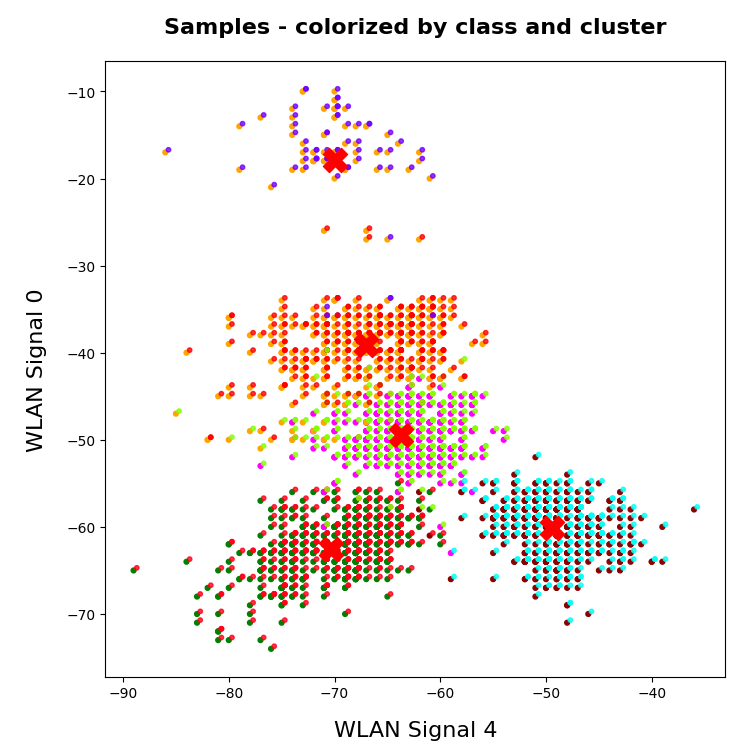We see that the border of the class related points on average overlaps well with the border of the clusters. Only a few points show a major deviation.

Is this always the case? Of course not!

We see this already when we we just use 2 clusters and colorize the data points according to cluster membership in one of the two clusters: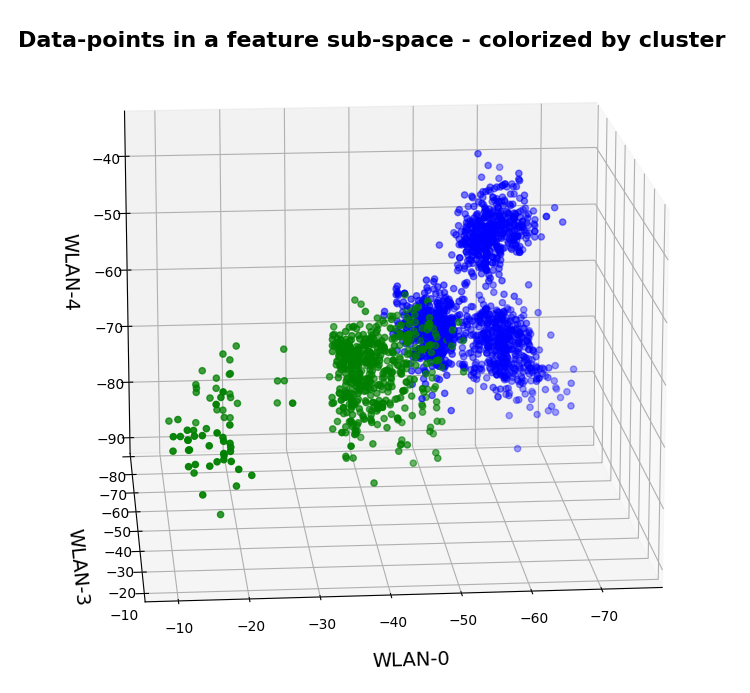Oops, all the beauty is gone! Members of the previously visual dark-red, green and pink „clusters“ would now certainly be members of the first defined „green“ cluster. And members of the visual pink and orange clusters would now be members of the „defined“ blue cluster. So: Labels may but do strong>not always define clusters.

An important thing we learned by this consideration is that we should at least use a number of cluster greater or equal the number of labels.

But even then the separation may not work. Let us imagine three rooms: In room „A“ we only have male persons, in room „B“ only female persons and in the third room „C“ female persons on one side of the room and male persons on the other – like in some old fashioned dancing courses. If we used the persons‘ coordinates to define features and the „male“/“female“ attributes as labels and tried a cluster analysis in the feature space we would clearly identify three clusters. However, in the cluster for room „C“ we would find a mixture of samples with different labels. Spatial vicinity in the feature space does not mean class identity and a label does not necessarily mean a big distance in the feature space.

# Hyperplanes and clusters

The only thing you can hope for with respect to a solvable ML problem is that the data points for different labels may be distributed such that a complicated and curved hyperplane can be found in the multidimensional feature space which separates groups of data points with the same label sufficiently well. But this hyperplane does not necessarily coincide with fictitious borders of some clusters identified by KMeans. We are lucky if the topologically closed surface of a cluster lies completely in a region of the hyperspace separated by complex and topologically open hyperplanes separating data points belonging to one class from other feature space regions which contain data points with different labels.

Not all data ensembles in ML will fall apart in extended clusters each of which dominated by some specific label. Ring like distributions of data samples with identical labels may pose a problem to cluster algorithms – mot only in 2 dimensions.

On the other side: If the labeled data – after some useful transformation – are not well separable in the feature space, then the posed ML task is problematic anyway and the chosen feature definitions may not be appropriate.

# Granularity: Hope for label dominance in sufficiently fine grained clusters

What does a label-cluster correlation depend on? Well, if there is an important factor regarding the position of the centroids of KMeans then it is the number of clusters. In the extreme case of as many clusters as data points a super-well defined cluster/label-association exists – but it is not of much use for ML-task. It just represents the most extreme case of overfitting you can think of. It will be useless for new unknown samples.

But the example with the distribution of male/female samples gives you an indication of what we should try: With a growing number of cluster the chances for a fine grained separation into clusters residing on one side of a hyperplane separating samples of different labels rises and with it the chance for a clearer dominance of one label per cluster. This is even true for ring like data distributions: With more then 4 clusters we may even describe a ring like data distribution quite well.

Therefore: If the sample distribution has some reasonable separation hyperplane at all there is a chance that you may find a number of clusters for which each cluster is dominated by a specific label.

In the case of the WIFI example this is pretty obvious. The following first plot shows the data points colorized according to their labels:

The next plot shows four cluster – with data points colorized according to their cluster membership: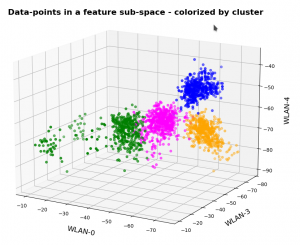The colors are different (we have no control of it) – but the different data point „clouds“ in the pictures coincide rather well. Only some data points do not behave well. We can again use the trick we iused in 2 dimensions and superimpose data points with colorization according to cluster and class: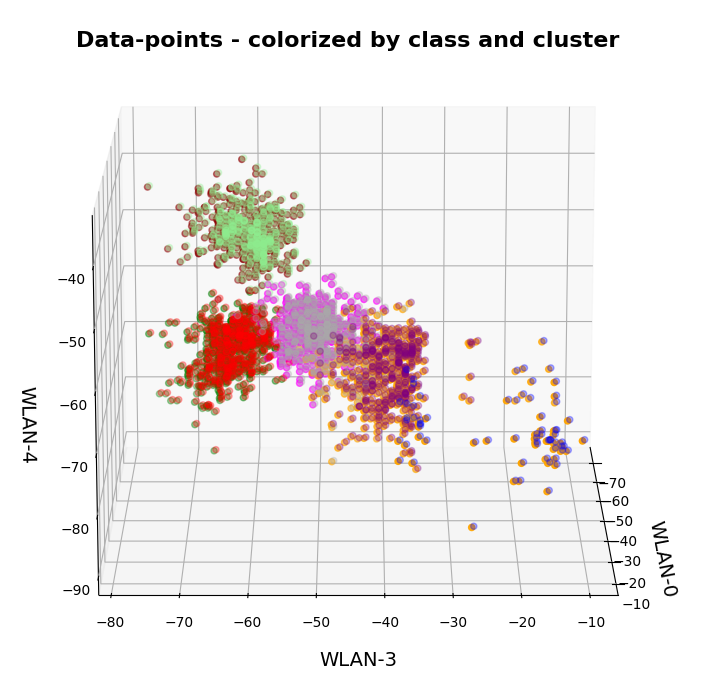# How do we define a classifier by the help of KMeans?

We need a predict()-function which predicts a label from a predicted cluster membership. The recipe is rather simple:

Define the number of cluster you want to use.

• Use KMeans for a cluster analysis of a training set of samples.
• Predict the cluster-membership of a sample with the help of the fitted kmeans-object and its predict()-function.
• Get the labels of the samples belonging to a specific cluster.
• Find out what the amount of samples with a certain label for a cluster is and compared the data.
• Find the label which contributes most samples to a cluster. Check the relative amount of deviating data points with other labels. Should be sufficiently small…
• Build a dictionary which associates the cluster number with its dominant label.
• Build a prediction function for yet unknown data points. It first predicts the cluster and then the associated label.

You should then test the accuracy of your new cluster-based classifier model on test data.

# More clusters than labels?

What will happen to such an algorithm if you use more clusters than labels? Short answer: Nothing – as long as each cluster is really dominated by a specific label. More precisely: A vast majority of your clusters should exhibit contributions from data points with one specific label to an amount significantly far beyond the statistical average. Having more clusters means that under reasonable conditions we just have more than one cluster predicting a certain label. For the case of the WIFI example this means that we can work with five clusters without getting nervous.

# Application to the WIFI example

As the clusters represent the labels quite well we expect very good values for the recall values. The experiment is pretty simple. I just present the resulting confusion matrices for the four labels (identifying rooms) and different numbers of clusters „nclus“. I first show you a presentation with seaborn, which explains, how to interpret rows and columns:

Here I used five (5) clusters and included all samples in the calculation. I.e. I did not separate a training set from a test set of data points.

# Confusion matrices for different numbers of clusters

nclus = 4

```[[496   0   4   0]
[  0 425  75   0]
[  2   0 492   6]
[  2   0   2 496]]
num of wrongly predicted samples:  91  :: avg_err =  0.0455
```

The „avg_err“ gives you the number of wrongly predicted samples divided by the total number of samples. We see that 4 clusters have a problem with the differentiation of the data points for the room „Diele“.

nclus = 5

```[[495   0   5   0]
[  0 455  45   0]
[  2   0 493   5]
[  2   0   2 496]]
num of wrongly predicted samples:  61  :: avg_err =  0.0305
```

nclus = 6

```[[499   0   1   0]
[  0 452  48   0]
[  5   0 490   5]
[  2   0   2 496]]
num of wrongly predicted samples:  63  :: avg_err =  0.0315
```

nclus = 7

```[[499   0   1   0]
[  0 492   8   0]
[  4  38 455   3]
[  2   0   3 495]]
num of wrongly predicted samples:  59  :: avg_err =  0.0295
```

nclus =

```num wrongly predicted samples:  58  :: avg_err =  0.029
```

nclus = 9

```[[499   0   1   0]
[  0 484  16   0]
[  4  16 476   4]
[  2   0   2 496]]
num of wrongly predicted samples:  45  :: avg_err =  0.0225
```

All data above were derived for the same random_state-variable to KMeans.

However, this result depends on the initial shuffling of the samples, the random_state parameter and the initial statistical distribution of the centroids by KMeans. And other hyperparameters … The next plot shows a slightly different result for nclus = 9:

This means: Nine cluster give us a reasonably fine grained resolution in the case of the WIFI example.

But: Note that the problem areas in the confusion matrix have changed. „Diele“ is handled a bit better, but „Wohnzimmer“ is not so sharply separated as before. This is not astonishing as we saw a significant mix of data of different labels for different clusters above already: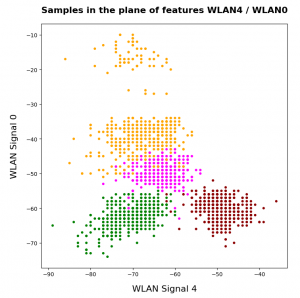After nclus = 9 we do not get much better – and dance around an average error of 0.025 => 2.5%:

nclus = 10

```num of wrongly predicted samples:  55  :: avg_err =  0.0275
```

nclus = 11

```num of wrongly predicted samples:  52  :: avg_err =  0.026
```

nclus = 25

```num of wrongly predicted samples:  40  :: avg_err =  0.02
```

Being conservative we can say that our simple cluster based classifier approaches an accuracy around 97.4%. Not so bad regarding the crudeness of our approach! A random forest algorithm reaches something above 98.2%. This is not so much better.

# Results after a separation of test data samples

I divided the data set than into 1500 train and 500 test samples.

For 9 clusters I got:

nclus = 9

```[[374   0   1   0]
[  0 362  13   0]
[  3  17 352   3]
[  2   0   2 371]]
num wrongly predicted train samples:  41  :: avg_err =  0.027333333333333334
num wrongly predicted train samples:  8  :: avg_err =  0.016
```

But these numbers depend strongly on the splitting – even if we split stratified. Another run gives:

nclus = 9

```[[374   0   1   0]
[  0 352  23   0]
[  3   0 369   3]
[  1   0   2 372]]
num wrongly predicted train samples:  33  :: avg_err =  0.022
num wrongly predicted test samples:  11  :: avg_err =  0.022
```

Other runs may even give higher average error values. The variation depend upon of how many critical data points in the intermixing zone were omitted in the train samples. At least the results are not too different from the ones named above.

# Conclusion

In this article I presented a very simple method by which a cluster algorithm can be used as a classifier. When we applied the approach to the rather simple WIFI example we saw that this worked pretty well.

What we in addition should try is to combine the classifier with a dimension reduction based on a PCA-analysis. From the results of a previous post we would expect that a cluster classifier should work well on the WIFI data after a transformation and projection of the samples‘ data points into a 3-dimensional space spanned by the most important orthogonal main component axes. This is the topic of the next post in this series:

Ceterum censeo: The worst fascist today who must be denazified is the Putler.

# A simple CNN for the MNIST dataset – VI – classification by activation patterns and the role of the CNN’s MLP part

I continue with my series on a simple CNN used upon the MNIST dataset.

In the last article I discussed the following points:

• The series of convolutional transformations, which a CNN applies to its input, eventually leads to abstract representations in low dimensional parameter spaces, called maps. In the case of our CNN we got 128 (3×3)-maps at the last convolutional layer. 3×3 indeed means a very low resolution.
• We saw that the transformations would NOT produce results on the eventual maps which could be interpreted in the sense of figurative elements of depicted numbers, such as straight lines, circles or bows. Instead, due to pooling layers, lines and curved line elements obviously experience a fast dissolution during propagation through the various Conv layers. Whilst the first Conv layer still gives fair representations of e.g. a „4“, line-like structures get already unclear at the second Conv layer and more or less disappear at the maps of the last convolutional layer.
• This does not mean that a map on a deep convolutional layer does not react to some specific pattern within the pixel data of an input image. We called such patterns OIPs in last article and we were careful to describe them as geometrical correlations of pixels – and not conceptual entities. The sequence of convolutions which makes up a map on a deep convolutional layer corresponds to a specific combination of filters applied to the image data. This led us to the the theoretical idea that a map may indeed select a specific OIP in an input image and indicate the existence of such a OIP pattern by some activation pattern of the „neurons“ within the map. However, we have no clue at the moment what such OIPs may look like and whether they correspond to conceptual entities which other authors usually call „features“.
• We saw that the common elements of the maps of multiple images of a handwritten „4“ correspond to point-like activations within specific low dimensional maps on the output side of the last convolutional layer.
• The activations seem to form abstract patterns across the maps of the last convolutional layer. These patterns, which we called FCPs, seem to support classification decisions, which the MLP-part of the CNN has to make.

So, at our present level of the analysis of a CNN, we cannot talk in a well founded way about „features“ in the sense of conceptual entities. We got, however, the impression that eventual abstractions of some patterns which are present in MNIST images of different digits lead to FCP patterns across maps which
allow for a classification of the images (with respect to the represented digits). We identified at least some common elements across the eventual maps of 3 different images of handwritten „4“s.

But it is really this simple? Can we by just looking for visible patterns in the activation output of the last convolutional layer already discriminate between different digits?

In this article I want to show that this is NOT the case. To demonstrate this we shall look at the image of a „4“ which could also be almost classified to represent a „9“. We shall see

• that the detection of clear unique patterns becomes really difficult when we look at the representations of „4“s which almost resemble a „9“ – at least from a human point of view;
• that directly visible patterns at the last convolutional layer may not contain sufficiently clear information for a classification;
• that the MLP part of our CNN nevertheless detects patterns after a linear transformation – i.e. after a linear combination of the outputs of the last Conv layer – which are not directly evident for human eyes. These „hidden“ patterns do, however, allow for a rather solid classification.

# What have „4“s in common after three convolutional transformations?

As in the last article I took three clear „4“ images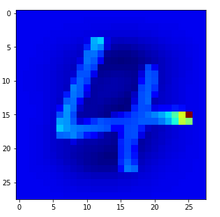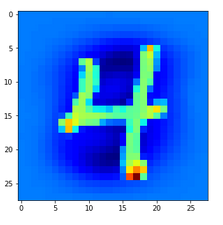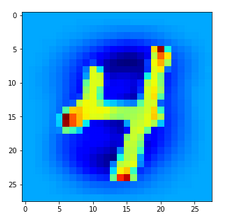and compared the activation output after three convolutional transformations – i.e. at the output side of the last Conv layer which we named „Conv2D_3“: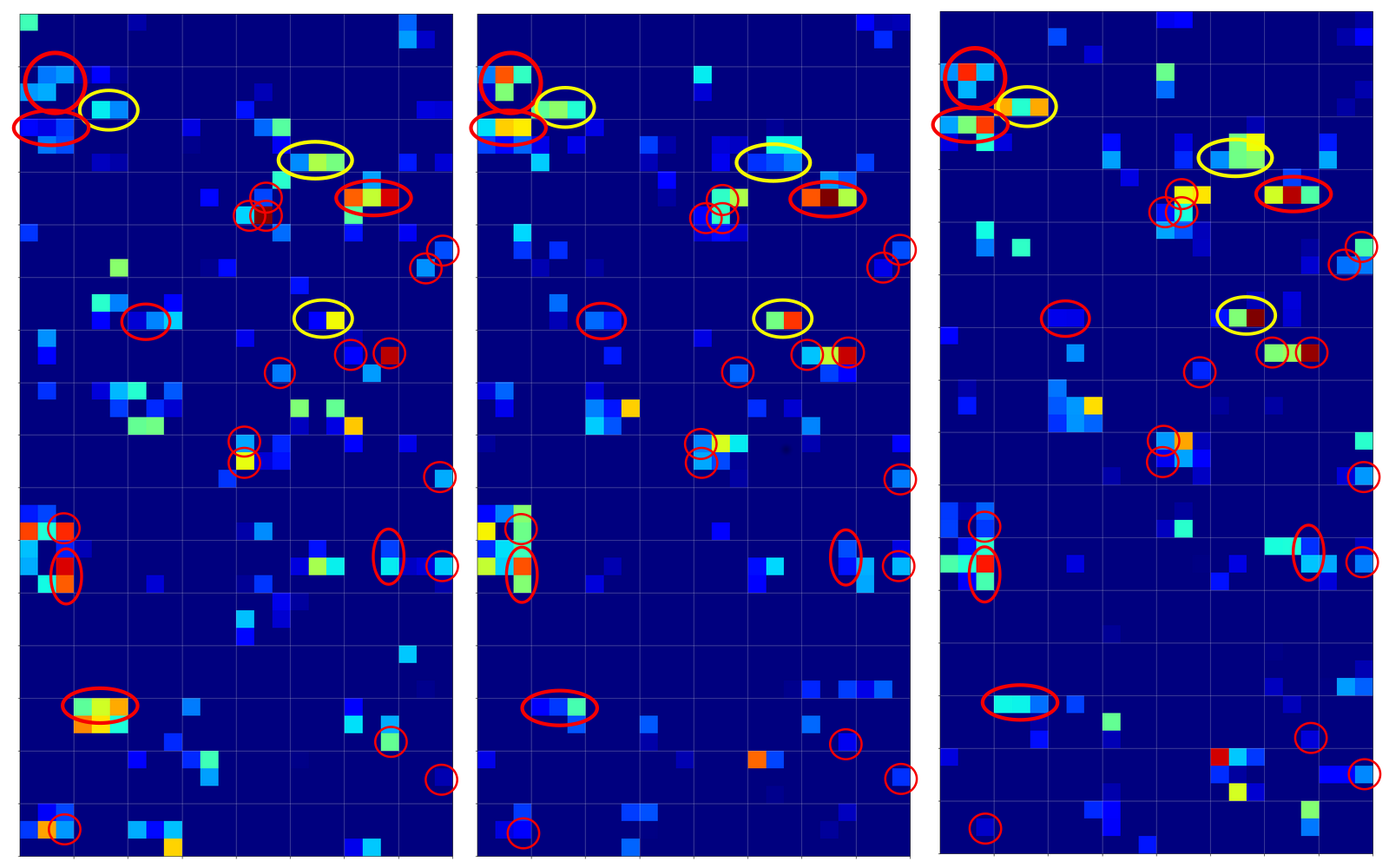The red circles indicate common points in the resulting 128 maps which we do not find in representations of three clear „9“s (see below). The yellow circles indicate common patterns which, however, appear in some representations of a „9“.

# What have „9“s in common after three convolutional transformations?

Now let us look at the same for three clear „9“s: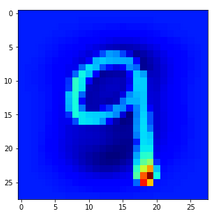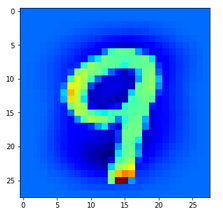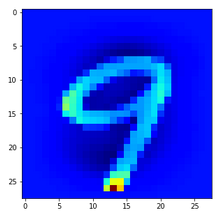A comparison gives the following common features of „9“s on the third Conv2D layer: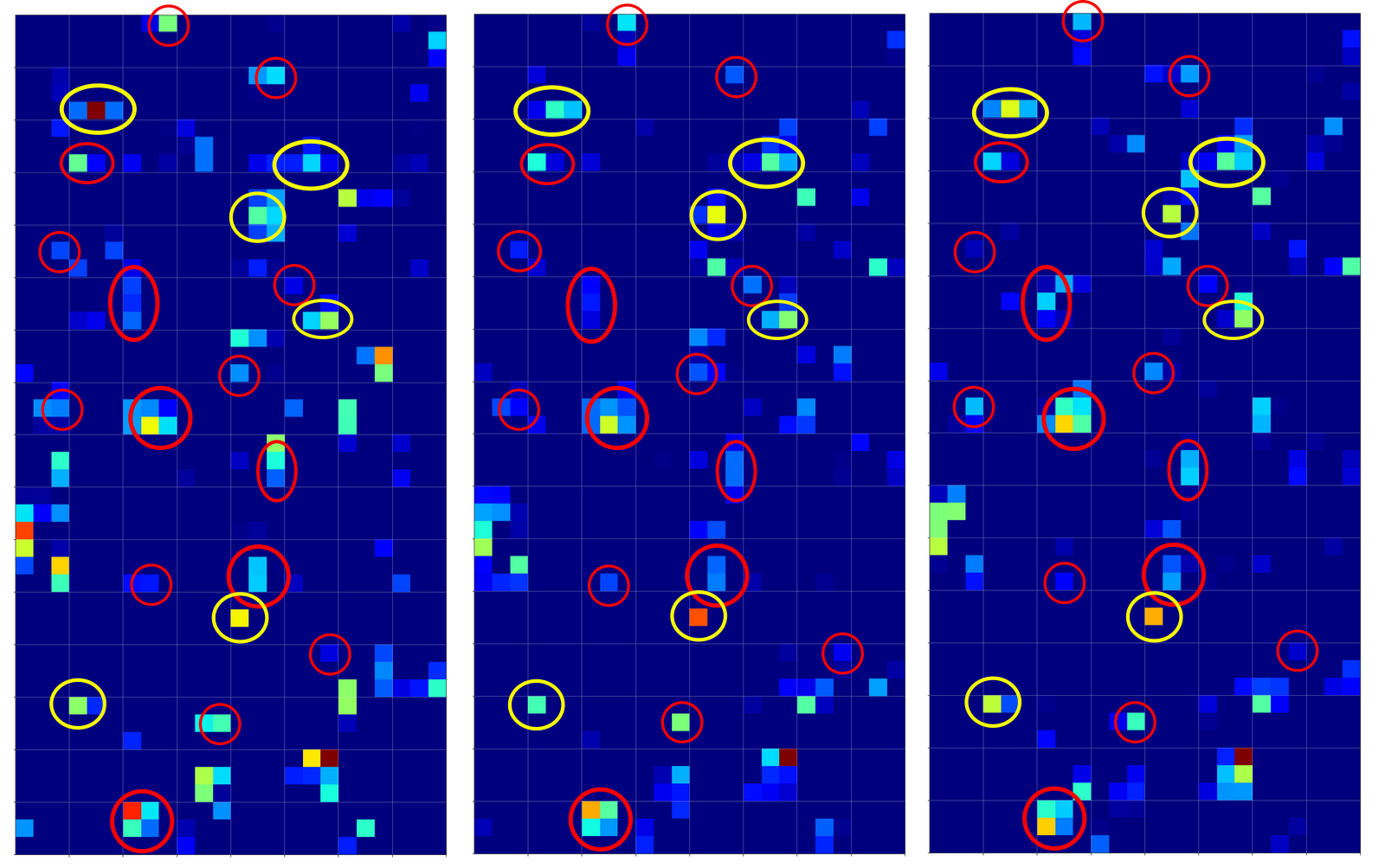We again get the impression that enough unique features seem to exist on the maps for „4“s and „9“s, respectively, to distinguish between images of these numbers. But is it really so simple?

# Intermezzo: Some useful steps to reproduce results

You certainly do not want to perform a training all the time when you want to analyze predictions at certain layers for some selected MNIST images. And you may also need the same „X_train“, „X_test“ sets to identify one and the same image by a defined number. Remember: In the Python code which I presented in a previous article for the setup for the data samples no unique number would be given due to initial shuffling.

Thus, you may need to perform a training run and then save the model as well as your X_train, y_train and X_test, y_test datasets. Note that we have transformed the data already in a reasonable tensor form which Keras expects. We also had already used one-hot-labels. The transformed sets were called „train_imgs“, „test_imgs“, „train_labels“, „test_labels“, „y_train“, „y_test“

The following code saves the model (here „cnn“) at the end of a training and loads it again:

```# save a full model
cnn.save('cnn.h5')

```

On a Linux system the default path is typically that one where you keep your Jupyter notebooks.

The following statements save the sets of tensor-like image data in Numpy compatible data (binary) structures:

```# Save the data

from numpy import save
save('train_imgs.npy', train_imgs)
save('test_imgs.npy', test_imgs)
save('train_labels.npy', train_labels)
save('test_labels.npy', test_labels)
save('y_train.npy', y_train)
save('y_test.npy', y_test)
```

```# Load train, test image data (in tensor form)

```

Be careful to save only once – and not to set up and save your training and test data again in a pure analysis session! I recommend to use different notebooks for training and later analysis. If you put all your code in just one notebook you may accidentally run Jupyter cells again, which you do not want to run during analysis sessions.

# What happens for unclear representations/images of a „4“?

When we trained a pure MLP on the MNIST dataset we had a look at the confusion matrix:
A simple Python program for an ANN to cover the MNIST dataset – XI – confusion matrix.
We saw that the MLP e.g. confused „5“s with „9s“, „9“s with „4“s, „2“s with „7“s, „8“s with „5“s – and vice versa. We got the highest confusion numbers for the misjudgement of badly written „4“s and „9“s.

Let us look at a regular 4 and two „4“s which with some good will could also be interpreted as representations of a „9“; the first one has a closed upper area – and there are indeed some representations of „9“s in the MNIST dataset which look similar. The second „4“ in my view is even closer to a „9“: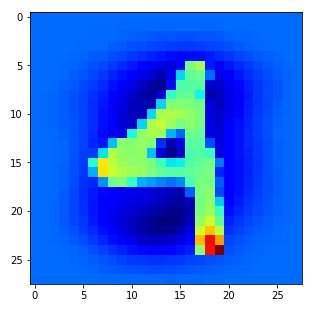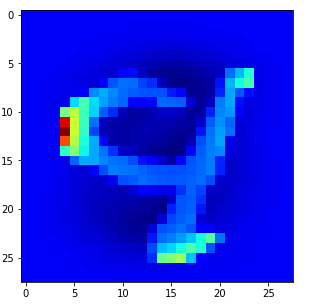Now, if we wanted to look out for the previously discussed „unique“ features of „4“s and „9s“ we would get a bit lost: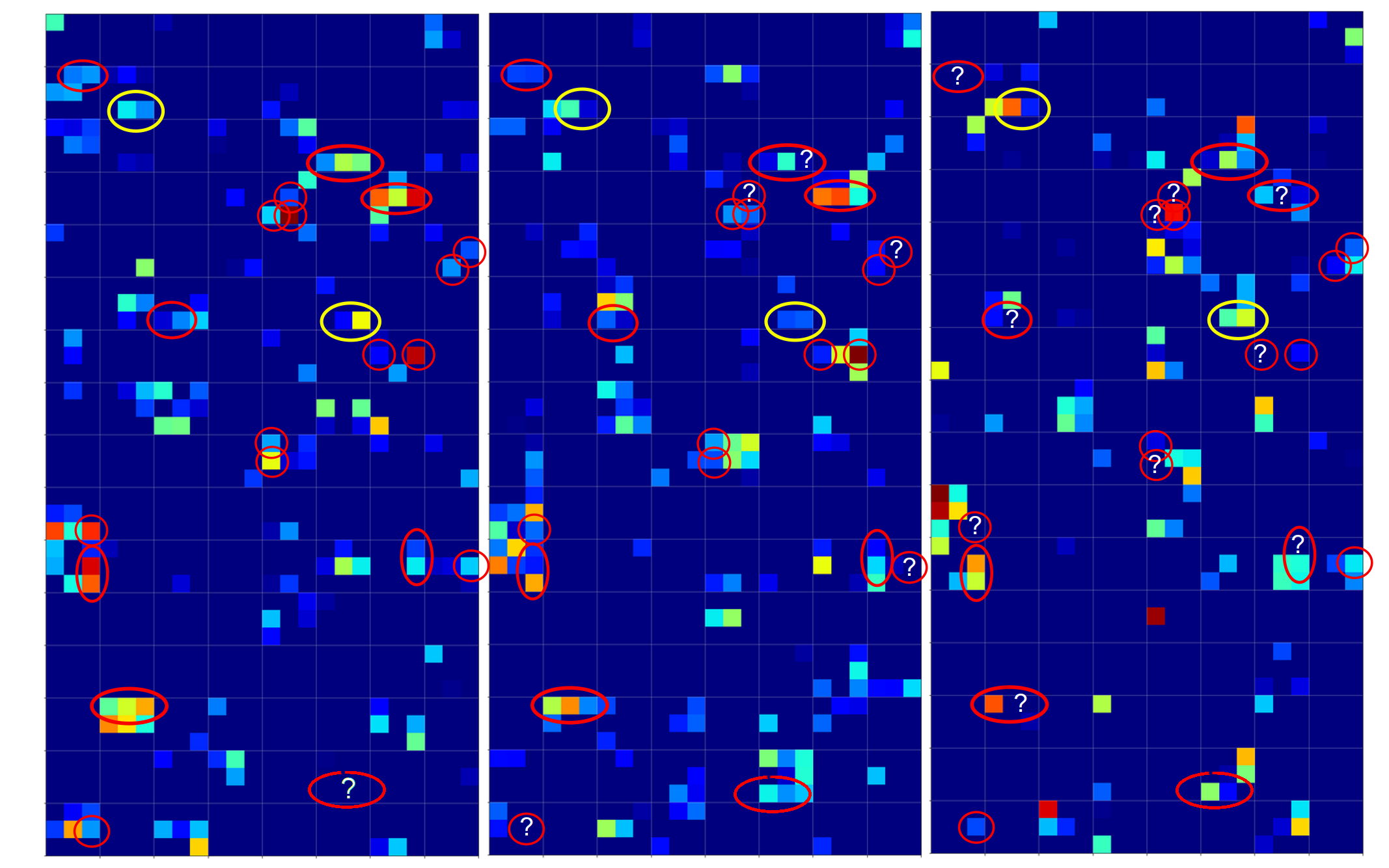The first image is for a clear „4“. The last two are the abstractions for our two newly chosen unclear „4“s in the order given above.

You see: Many of our seemingly „unique features“ for a „4“ on the third Conv-level are no longer or not fully present for our second „4“; so we would be rather insecure if we had to judge the abstraction as a viable pattern for a „4“. We would expect that this „human“ uncertainty also shows up in a probability distribution at the output layer of our CNN.

But, our CNN (including its MLP-part) has no doubt about the classification of the last sample as a „4“. We just look at the prediction output of our model:

```# Predict for a single image
# ****************************
num_img = 1302
ay_sgl_img = test_imgs[num_img:num_img+1]
print(ay_sgl_img.shape)
# load last cell for the next statement to work
#prob = cnn_pred.predict_proba(ay_sgl_img, batch_size=1)
#print(prob)
prob1 = cnn_pred.predict(ay_sgl_img, batch_size=1)
print(prob1)

[[3.61540742e-07 1.04205284e-07 1.69877489e-06 1.15337198e-08
9.35641170e-01 3.53500056e-08 1.29525617e-07 2.28584581e-03
2.59062881e-06 6.20680153e-02]]

```

93.5% probability for a „4“! A very clear discrimination! How can that be, given the – at first sight – seemingly unclear pattern situation at the third activation layer for our strange 4?

# The MLP-part of the CNN „sees“ things we humans do not see directly

We shall not forget that the MLP-part of the CNN plays an important role in our game. It reduces the information of the last 128 maps (3x3x128 = 1152) values down to 100 node values with the help of 115200 distinguished weights for related connections. This means there is a lot of fine-tuned information extraction and information compactification going on at the border of the CNN’s MLP part – a transformation step which is too complex to grasp directly.

It is the transformation of all the 128x3x3-map-data into all 100 nodes via a linear combination which makes things difficult to understand. 115200 optimized weights leave enough degrees of freedom to detect combined patterns in the activation data which are more complex and less obvious than the point-like structures we encircled in the images of the maps

So, it is interesting to visualize and see how the MLP part of our CNN reacts to the activations of the last convolutional layers. Maybe we find some more intriguing patterns there, which discriminate „4“s from „9“s and explain the rather clear probability evaluation.

# Visualization of the output of the dense layers of the CNN’s MLP-part

We need to modify some parts of our code for creating images of the activation outputs of convolutional layers to be able to produce equally reasonable images for the output of the dense MLP layers, too. These modifications are simple. We distinguish between the types of layers by their names: When the name contains „dense“ we execute a slightly different code. The changes affect just the function „img_grid_of_layer_activation()“ previously discussed as the contents of a Jupyter „cell 9„:

```
# Function to plot the activations of a layer
# --------------------------
-----------------
# Adaption of a method originally designed by F.Chollet

def img_grid_of_layer_activation(d_img_sets, model_fname='cnn.h5', layer_name='', img_set="test_imgs", num_img=8,
scale_img_vals=False):
'''
Input parameter:
-----------------
d_img_sets: dictionary with available img_sets, which contain img tensors (presently: train_imgs, test_imgs)
model_fname: Name of the file containing the models data
layer_name: name of the layer for which we plot the activation; the name must be known to the Keras model (string)
image_set: The set of images we pick a specific image from (string)
num_img: The sample number of the image in the chosen set (integer)
scale_img_vals: False: Do NOT scale (standardize) and clip (!) the pixel values. True: Standardize the values. (Boolean)

Hints:
-----------------
We assume quadratic images - in case of dense layers we assume a size of 1
'''

# get the output of a certain named layer - this includes all maps
# https://keras.io/getting_started/faq/#how-can-i-obtain-the-output-of-an-intermediate-layer-feature-extraction
cnnx_layer_output = cnnx.get_layer(layer_name).output

# build a new model for input "cnnx.input" and output "output_of_layer"
# ~~~~~~~~~~~~~~~~~
# Keras knows the required connections and intermediat layers from its tensorflow graphs - otherwise we get an error
# The new model can make predictions for a suitable input in the required tensor form
mod_lay = models.Model(inputs=cnnx.input, outputs=cnnx_layer_output)

# Pick the input image from a set of respective tensors
if img_set not in d_img_sets:
print("img set " + img_set + " is not known!")
sys.exit()
# slicing to get te right tensor
ay_img = d_img_sets[img_set][num_img:(num_img+1)]

# Use the tensor data as input for a prediction of model "mod_lay"
lay_activation = mod_lay.predict(ay_img)
print("shape of layer " + layer_name + " : ", lay_activation.shape )

# number of maps of the selected layer
n_maps   = lay_activation.shape[-1]
print("n_maps = ", n_maps)

# size of an image - we assume quadratic images
# in the case  of "dense" layers we assume that the img size is just 1 (1 node)
if "dense" in layer_name:
img_size = 1
else:
img_size = lay_activation.shape
print("img_size = ", img_size)

# Only for testing: plot an image for a selected
# map_nr = 1
#plt.matshow(lay_activation[0,:,:,map_nr], cmap='viridis')

# We work with a grid of images for all maps
# ~~~~~~~~~~~~~~~----------------------------
# the grid is build top-down (!) with num_cols and num_rows
# dimensions for the grid
num_imgs_per_row = 8
num_cols = num_imgs_per_row
num_rows = n_maps // num_imgs_per_row
#print("img_size = ", img_size, " num_cols = ", num_cols, " num_rows = ", num_rows)

# grid
dim_hor = num_imgs_per_row * img_size
dim_ver = num_rows * img_size
img_grid = np.zeros( (dim_ver, dim_hor) )   # horizontal, vertical matrix
print("shape of img grid = ", img_grid.shape)

# double loop to fill the grid
n = 0
for row in range(num_rows):
for col in range(num_cols):
n += 1
#print("n = ", n, "row = ", row, " col = ", col)
# in case of a dense layer the shape of the tensor like output
# is different in comparison to Conv2D layers
if "dense" in layer_name:
present_img = lay_activation[ :, row*num_imgs_per_row + col]
else:

present_img = lay_activation[0, :, :, row*num_imgs_per_row + col]

# standardization and clipping of the img data
if scale_img_vals:
present_img -= present_img.mean()
if present_img.std() != 0.0: # standard deviation
present_img /= present_img.std()
#present_img /= (present_img.std() +1.e-8)
present_img *= 64
present_img += 128
present_img = np.clip(present_img, 0, 255).astype('uint8') # limit values to 255

# place the img-data at the right space and position in the grid
# ~~~~~~~~~~~~~~~~~~~~~~~~~~~~~~~~~~~~~~~~~~~~~~~~~~~~~~~~~~~~~~
# the following is only used if we had reversed vertical direction by accident
#img_grid[row*img_size:(row+1)*(img_size), col*img_size:(col+1)*(img_size)] = np.flip(present_img, 0)
img_grid[row*img_size:(row+1)*(img_size), col*img_size:(col+1)*(img_size)] = present_img

return img_grid, img_size, dim_hor, dim_ver
```

You certainly detect the two small changes in comparison to the code for Jupyter cell 9 of the article
A simple CNN for the MNIST dataset – IV – Visualizing the output of convolutional layers and maps.

However, there remains one open question: We were too lazy in the coding discussed in previous articles to create our own names names for the dense layers. This is, however, no major problem: Keras creates its own names – if we do not define our own layer names when constructing a CNN model. Where do we get these default names from? Well, from the model’s summary:

```cnn_pred.summary()

Model: "sequential_7"
_________________________________________________________________
Layer (type)                 Output Shape              Param #
=================================================================
Conv2D_1 (Conv2D)            (None, 26, 26, 32)        320
_________________________________________________________________
Max_Pool_1 (MaxPooling2D)    (None, 13, 13, 32)        0
_________________________________________________________________
Conv2D_2 (Conv2D)            (None, 11, 11, 64)        18496
_________________________________________________________________
Max_Pool_2 (MaxPooling2D)    (None, 5, 5, 64)          0
_________________________________________________________________
Conv2D_3 (Conv2D)            (None, 3, 3, 128)         73856
_________________________________________________________________
flatten_7 (Flatten)          (None, 1152)              0
_________________________________________________________________
dense_14 (Dense)             (None, 100)               115300
_________________________________________________________________
dense_15 (Dense)             (None, 10)                1010
=================================================================
Total params: 208,982
Trainable params: 208,982
Non-trainable params: 0
_________________________________________________________________

```

Our first MLP layer with 100 nodes obviously got the name „dense_14“.

With our modification and the given name we can now call Jupyter „cell 10“ as before:

```
# Plot the img grid of a layers activation
# ~~~~~~~~~~~~~~~~~~~~~~~~~~~~~~~~~~~~~~~~~

# global dict for the image sets
d_img_sets= {'train_imgs':train_imgs, 'test_imgs':test_imgs}

# layer - pick one of the names which you defined for your model
layer_name = "dense_14"

# choose a image_set and an img number
img_
set = "test_imgs"

# clear 4
num_img = 1816

#unclear 4
#num_img = 1270
#num_img = 1302

#clear 9
#num_img = 1249
#num_img = 1410
#num_img = 1858

# Two figures
# -----------
fig1 = plt.figure(1, figsize=(5,5))  # figure for the input img
fig2 = plt.figure(2)  # figure for the activation outputs of th emaps

fig1 = plt.figure( figsize=(5,5) )
ay_img = test_imgs[num_img:num_img+1]
#plt.imshow(ay_img[0,:,:,0], cmap=plt.cm.binary)
plt.imshow(ay_img[0,:,:,0], cmap=plt.cm.jet)

# getting the img grid
img_grid, img_size, dim_hor, dim_ver = img_grid_of_layer_activation(
d_img_sets, model_fname='cnn.h5', layer_name=layer_name,
img_set=img_set, num_img=num_img,
scale_img_vals=False)
# Define reasonable figure dimensions by scaling the grid-size
scale = 1.6 / (img_size)
fig2 = plt.figure( figsize=(scale * dim_hor, scale * dim_ver) )
#axes
ax = fig2.gca()
ax.set_xlim(-0.5,dim_hor-1.0)
ax.set_ylim(dim_ver-1.0, -0.5)  # the grid is oriented top-down
#ax.set_ylim(-0,dim_ver-1.0) # normally wrong

# setting labels - tick positions and grid lines
ax.set_xticks(np.arange(img_size-0.5, dim_hor, img_size))
ax.set_yticks(np.arange(img_size-0.5, dim_ver, img_size))
ax.set_xticklabels([]) # no labels should be printed
ax.set_yticklabels([])

# preparing the grid
plt.grid(b=True, linestyle='-', linewidth='.5', color='#ddd', alpha=0.7)

# color-map
#cmap = 'viridis'
#cmap = 'inferno'
cmap = 'jet'
#cmap = 'magma'

plt.imshow(img_grid, aspect='auto', cmap=cmap)
```

In the output picture each node will be represented by a colored rectangle.

# Visualization of the output for clear „4“s at the first dense MLP-layer

The following picture displays the activation values for three clear „4“s at the first dense MLP layer: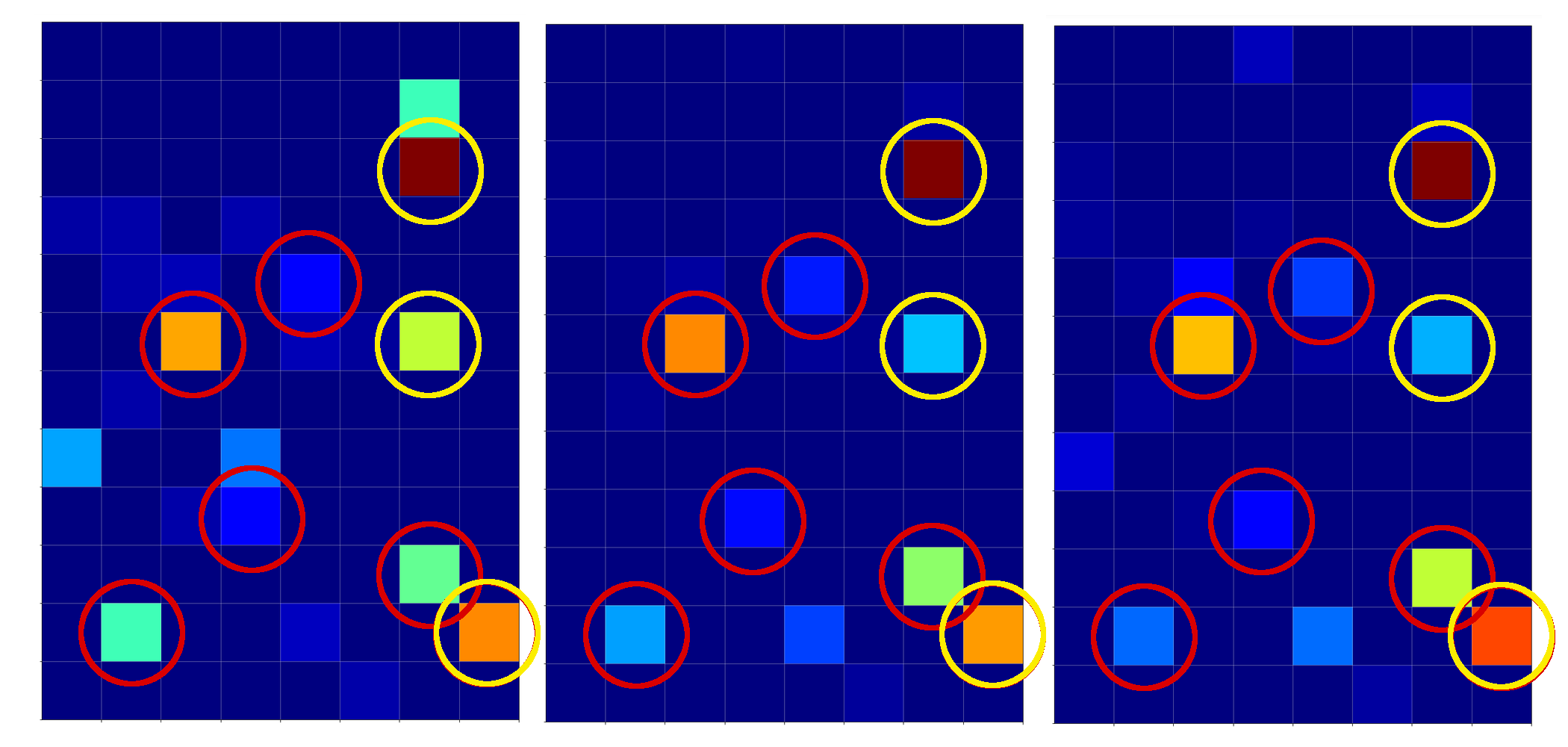I encircled again some of the nodes which carry some seemingly „unique“ information for representations of the digit „4“.

For clear „9“s we instead get: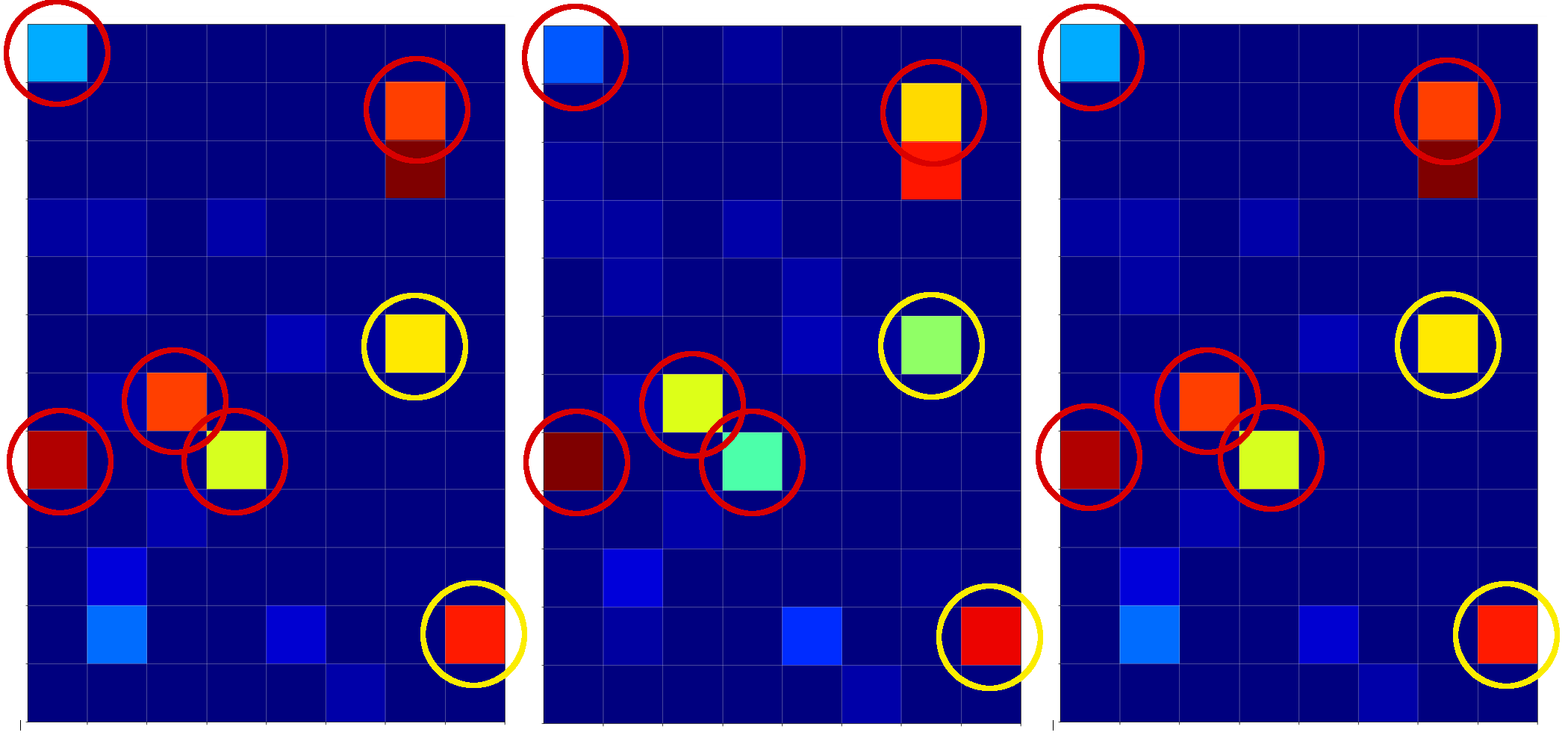Hey, there are some clear differences: Especially, the diagonal pattern (vertically a bit below the middle and horizontally a bit to the left) and the activation at the first node (upper left) seem to be typical for representations of a „9“.

# Our unclear „4“ representations at the first MLP layer

Now, what do we get for our two unclear „4“s?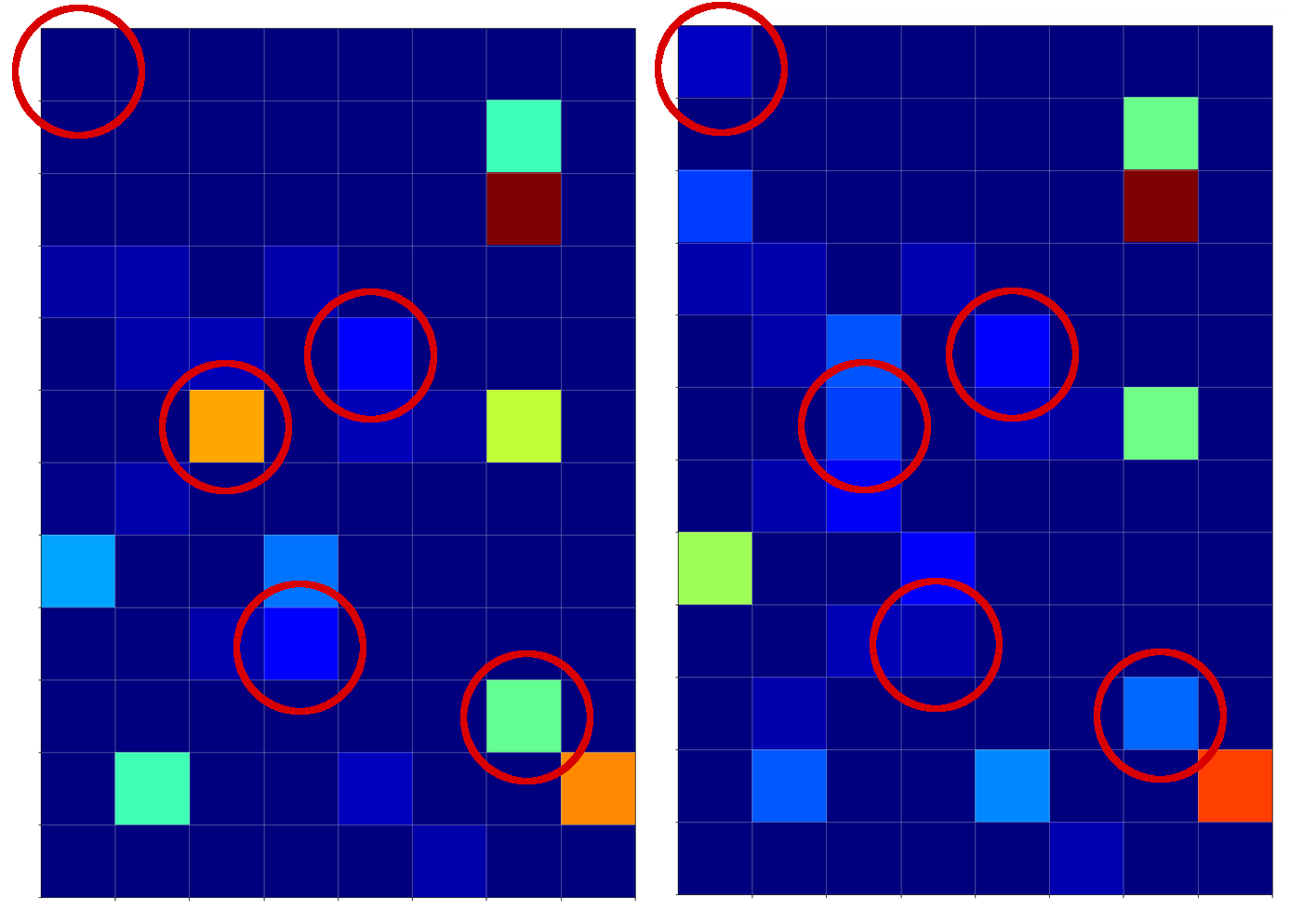I think that we would guess with confidence that our first image clearly corresponds to a „4“. With the second one we would be a bit more careful – but the lack of the mentioned diagonal structure with sufficiently high values (orange to yellow on the „jet“-colormap) would guide us to a „4“. Plus the presence of a relatively high value at a node present at the lower right which is nowhere in the „9“ representations. Plus too small values at the upper left corner. Plus some other aspects – some nodes have a
value where all the clear „9“s do not have anything.

We should not forget that there are more than 1000 weights again to emphasize some combinations and suppress others on the way to the output layer of the CNN’s MLP part.

# Conclusion

Information which is still confusing at the last convolutional layer – at least from a human visual perspective – can be „clarified“ by a combination of the information across all (128) maps. This is done by the MLP transformations (linear matrix plus non-linear activation function) which produce the output of the 1st dense layer.

Thus and of course, the dense layers of the MLP-part of a CNN play an important role in the classification process: The MLP may detect patterns in the the combined information of all available maps at the last convolutional layer which the human eye may have difficulties with.

In the sense of a critical review of the results of our last article we can probably say: NOT the individual points, which we marked in the images of the maps at the last convolutional layer, did the classification trick; it was the MLP analysis of the interplay of the information across all maps which in the end lead the CNN to an obviously correct classification.

Common features in calculated maps for MNIST images are nice, but without an analysis of a MLP across all maps they are not sufficient to solve the classification problem. So: Do not underestimate the MLP part of a CNN!

In the next article

A simple CNN for the MNIST dataset – VII – outline of steps to visualize image patterns which trigger filter maps

I shall outline some required steps to visualize the patterns or structures within an input image which a specific CNN map reacts to. This will help us in the end to get a deeper understanding of the relation between FCPs and OIPs. I shall also present some first images of such OIP patterns or „features“ which activate certain maps of our trained CNN.

# A single neuron perceptron with sigmoid activation function – I – failure of gradient descent due to saturation

Readers who follow my series on a Python program for a „Multilayer Perceptron“ [MLP] have noticed that I emphasized the importance of a normalization of input data in my last article:

A simple Python program for an ANN to cover the MNIST dataset – XII – accuracy evolution, learning rate, normalization

Our MLP „learned“ by searching for the global minimum of the loss function via the „gradient descent“ method. Normalization seemed to improve a smooth path to convergence whilst our algorithm moved into the direction of a global minimum on the surface of the loss or cost functions hyperplane over the weight parameter space. At least in our case where we used the sigmoid function as the activation function of the artificial neurons. I indicated that the reason for our observations had to do with properties of this function – especially for neurons in the first hidden layer of an MLP.

In case of the 4-layer MLP, which I used on the MNIST dataset, I applied a special form of normalization namely „standardization„. I did this by using the StandarScaler of SciKit-Learn. See the following link for a description: Sklearn preprocessing StandardScaler

We saw the smoothing and helpful impact of normalization on the general convergence of gradient descent by the help of a few numerical experiments. The interaction of the normalization of 784 features with mini-batches and with a complicated 4 layer-MLP structure, which requires the determination of several hundreds of thousands weight values is however difficult to grasp or analyze. One understands that there is a basic relation to the properties of the activation function, but the sheer number of the dimensions of the feature and weight spaces and statistics make a thorough understanding difficult.

Since then I have thought a bit about how to set up a really small and comprehensible experiment which makes the positive impact of normalization visible in a direct and visual form. I have come up with the following most simple scenario, namely the training of a simple perceptron with only one computing neuron and two additional „stupid“ neurons in an input layer which just feed input data to our computing neuron: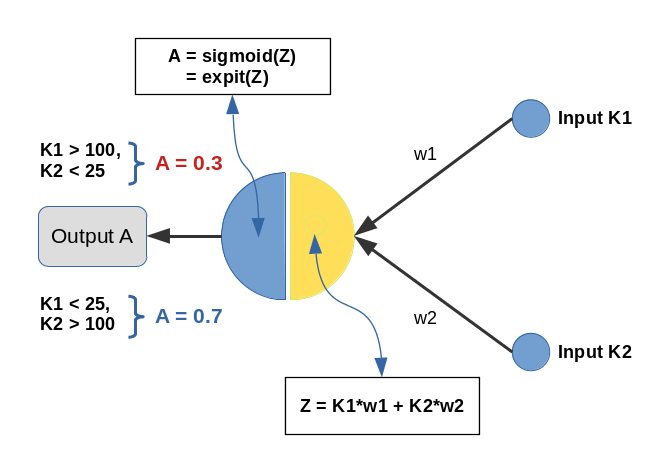Input values K1 and K2 are multiplied by weights w1 and w2 and added by the central solitary „neuron“. We use the sigmoid function as the „activation function“ of this neuron – well anticipating that the properties of this function may lead to trouble.

The perceptron has only one task: Discriminate between two different types of input data by assigning them two distinct output values.

• For K1 > 100 and K2 < 25 we want an output of A=0.3.
• For K1 &l; 25 and K2 > 100 we, instead, want an output of A=0.7

We shall feed the perceptron only 14 different pairs of input values K1[i], K2[i] (i =0,1,..13), e.g. in form of lists:

```li_K1 = [200.0,   1.0, 160.0,  11.0, 220.0,  11.0, 120.0,  22.0, 195.0,  15.0, 130.0,   5.0, 185.0,  16.0]
li_K2 = [ 14.0, 107.0,  10.0, 193.0,  32.0, 178.0,   2.0, 210.0,  12.0, 134.0,  15.0, 167.0,  10.0, 229.0]
```

(The careful reader detects one dirty example li_K2 = 32 (> 25), in contrast to our setting. Just
to see how much of an impact such a deviation has …)

We call each pair (K1, K2)=(li_K1[i], li_K2[i]) for a give „i“ a „sample„. Each sample contains values for two „features„: K1 and K2. So, our solitary computing neuron has to solve a typical classification problem – it shall distinguish between two groups of input samples. In a way it must learn the difference between small and big numbers for 2 situations appearing at its input channels.

Off topic: This morning I listened to a series of comments of Mr. Trump during the last weeks on the development of the Corona virus crisis in the USA. Afterwards, I decided to dedicate this mini-series of articles on a perceptron to him – a person who claims to „maybe“ be „natural talent“ on complicated things as epidemic developments. Enjoy (?) his own words via an audio compilation in the following news article:
https://www.faz.net/aktuell/politik/trumps-praesidentschaft/usa-zehn-wochen-corona-in-den-worten-von-trump-16708603.html

# Two well separable clusters

In the 2-dim feature space {K1, K2} we have just two clusters of input data: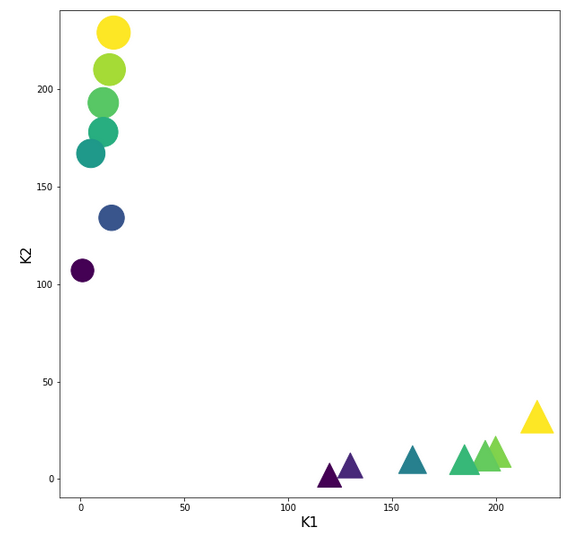Each cluster has a long diameter along one of the feature axes – but overall the clusters are well separable. A rather good separation surface would e.g. be a diagonal line.

For a given input sample with K-values K1 and K2 we define the output of our computing neuron to be

A(w1, w2) = expit( w1*K1 + w2*K2 ) ,

where expit() symbolizes the sigmoid function. See the next section for more details.

Corresponding target-values for the output A are (with at1 = 0.3 and at2 = 0.7):

```li_a_tgt = [at1,  at2,  at1,  at2,  at1,  at2,  at1,  at2,  at1,  at2,  at1,  at2,   at1,  at2]
```

With the help of these target values our poor neuron shall learn from the 14 input samples to which cluster a given future sample probably belongs to. We shall use the „gradient descent“ method to train the neuron for this classification task. o solve the task our neuron must find a reasonable separation surface – a line – in the {K1,K2}-plane; but it got the additional task to associate two distinct output values „A“ with the two clusters:

A=0.7 for data points with a large K1-value and A=0.3 for data points with a small K1-value.

So, the separation surface has to fulfill some side conditions.

Readers with a background in Machine Learning and MLPs will now ask: Why did you pick the special values 0.3 and 0.7? A good question – I will come back to it during our experiments. Another even more critical question could be: What about a bias neuron in the input layer? Don’t we need it? Again, a very good question! A bias neuron allows for a shift of a separation surface in the feature space. But due to the almost symmetrical nature of our input data (see the positions and orientations of the clusters!) and the target values the impact of a bias neuron on the end result would probably only be small – but we shall come back to the topic of a bias neuron in a later article. But you are free to extend the codes given below to account for a bias neuron in the input layer. You will notice a significant impact if you change either the relative symmetry of the input or of the output data. But lets keep things simple for the next hours …

# The sigmoid function and its saturation for big arguments

You can import the sigmoid function under the name „expit“ from the „scipy“ library into your Python code. The sigmoid function is a smooth one – but it quickly saturates for big negative or positive values: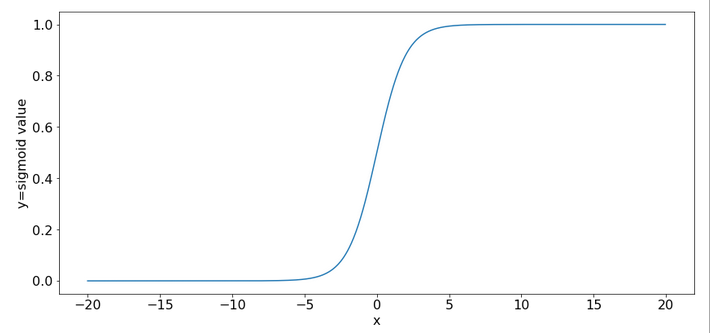So, output values get almost indistinguishable if the absolute values of the arguments are bigger than 15.

# What is interesting about our input data? What is the relation to MNIST data?

The special situation about the features in our example is the following: For one and the same feature we have a big number and a small number to work with – depending on the sample. Which feature value – K1 or K2 – is big depends on the sample.

This is something that also happens with the input „features“ (i.e. pixels) coming from a MNIST-image:
For a certain feature (= a pixel at a defined position in the 28×28 picture) in a given sample (= a distinct image) we may get a big number as 255. For another feature (= pixel) the situation may be totally different and we may find a value of 0. In another sample (= picture) we may get the reverse situation for the same two pixels.

What happens in such a situation at a specific neuron in the first hidden neuron layer of a MLP when we start gradient descent with a statistical distribution of weight values? If we are unlucky then the initial statistical weight constellation for a sample may lead to a big number of the total input to our selected hidden neuron with the effect of a very small gradient at this node – due to saturation of the sigmoid function.

To give you a feeling: Assume that you have statistical weights in the range of [-0.025, 0.025]. Assume further that only 4 pixels of a MNIST picture with a value of 200 contribute with a local maximum weight of 0.25; then we get a a minimum input at our node of 4*0.25*200 = 20. The gradient of expit(20) has a value of 2e-9. Even if we multiply by the required factor of 200 for a weight correction at one of the contributing input nodes we would would arrive at 4e-7. Quite hopeless. Of course, the situation is not that bad for all weights and image-samples, but you get an idea about the basic problem ….

Our simple scenario breaks the MNIST situation down to just two features and just one working neuron – and therefore makes the correction situation for gradient descent really extreme – but interesting, too. And we can analyze the situation far better than for a MLP because we deal with an only 2-dimensional feature-space {K1, K2} and a 2-dimensional weight-space {w1, w2}.

# A simple quadratic cost function for our neuron

For given values w1 and w2, i.e. a tuple (w1, w2), we define a quadratic cost or loss function C_sgl for one single input sample (K1, K2) as follows:

C_sgl = 0.5 * ( li_a_tgt(i) – expit(z_i) )**2, with z_i = li_K[i]*w1 + li_K2[i]*w2

The total cost-function for the batch of all 14 samples is just the sum of all these terms for the individual samples.

# Existence of a solution for our posed problem?

From all we theoretically know about the properties of a simple perceptron it should be able to find a reasonable solution! But, how do we know that a reasonable solution for a (w1, w2)-pair does exist at all? One line of reasoning goes like follows:

For two samples – each a member of either cluster – you can plot the hyperplanes of the related outputs „A(K1, K2) = expit(w1*K1+w2*K2)“ over the (w1, w2)-space. These hyperplanes are almost orthogonal to each other. If you project the curves of a cut with the A=0.3-planes and the A=0.7-planes down to the (w1, w2)-plane at the center you get straight
lines – almost orthogonally oriented against each other. So, such 2 specific lines cut each other in a well defined point – somewhere off the center. As the expit()-function is a relatively steep one for our big input values the crossing point is relatively close to the center. If we choose other samples we would get slightly different lines and different crossing points of the projected lines – but not too far from each other.

The next plot shows the expit()-functions close to the center of the (w1, w2)-plane for two specific samples of either cluster. We have in addition displayed the surfaces for A=0.7 and A=0.3.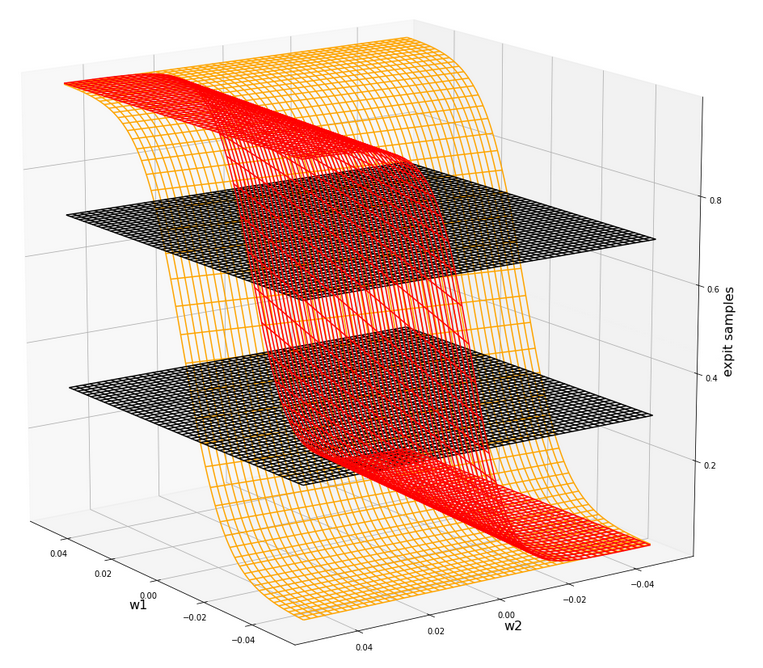The following plot shows the projections of the cuts of the surfaces for 7 samples of each cluster with the A=0.3-plane and the A=0.7, respectively.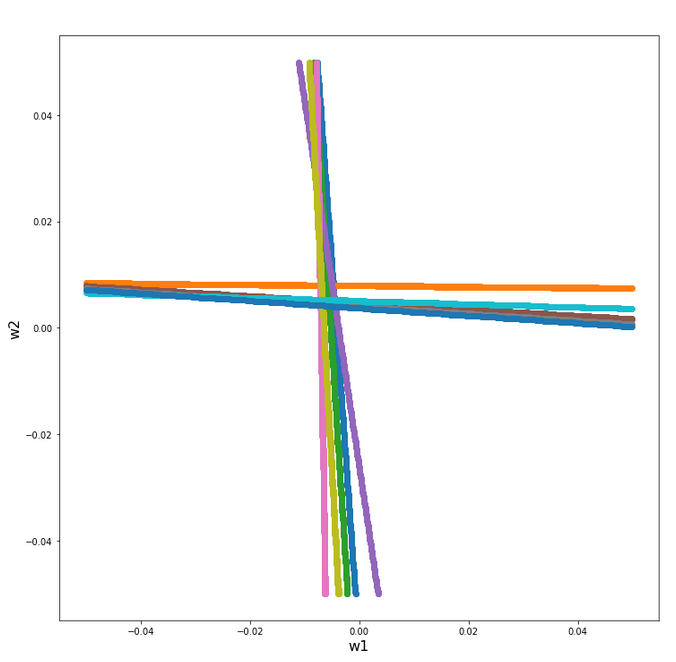The area of crossings is not too big on the chosen scale of w1, w2. Looking at the graphics we would expect an optimal point around (w1=-0.005, w2=+0.005) – for the original, unnormalized input data.

By the way: There is also a solution for at1=0.3 and at2=0.3, but a strange one. Such a setup would not allow for discrimination. We expect a rather strange behavior then. A first guess could be: The resulting separation curve in the (K1, K2)-plane would move out of the area between the two clusters.

# Code for a mesh based display of the costs over the weight-parameter space

Below you find the code suited for a Jupyter cell to get a mesh display of the cost values

```import numpy as np
import numpy as np
import random
import math
import sys

from sklearn.preprocessing import StandardScaler
from sklearn.preprocessing import Normalizer
from sklearn.preprocessing import MinMaxScaler
from scipy.special import expit

import matplotlib as mpl
from matplotlib import pyplot as plt
from matplotlib.colors import ListedColormap
import matplotlib.patches as mpat
from mpl_toolkits import mplot3d
from mpl_toolkits.mplot3d import Axes3D

# total cost functions for overview
# *********************************
def costs_mesh(num_samples, W1, W2, li_K1, li_K2, li_a_tgt):
zshape = W1.shape
li_Z_sgl = []
li_C_sgl = []
C = np.zeros(zshape)

rg_idx = range(num_samples)
for idx in rg_idx:
Z_idx      = W1 * li_K1[idx] + W2 * li_K2[idx]
A_tgt_idx  = li_a_tgt[idx] * np.ones(zshape)
C_idx = 0.5 * ( A_tgt_idx - expit(Z_idx) )**2
li_C_sgl.append( C_idx )
C += C_idx

C /= np.float(num_samples)
return C, li_C_sgl

# ******************
# Simple Perceptron
#*******************

# input at 2 nodes => 2 features K1 and K2 => there will be just one output neuron
li_K1 = [200.0,   1.0, 160.0,  11.0, 220.0,  11.0, 120.0,  14.0, 195.0,  15.0, 130.0,   5.0, 185.0,  16.0  ]
li_K2 = [ 14.0, 107.0,  10.0, 193.0,  32.0, 178.0,   2.0, 210.0,  12.0, 134.0,  7.0, 167.0,  10.0, 229.0 ]

# target values
at1 = 0.3; at2 = 0.7
li_a_tgt  = [at1,  at2,  at1,  at2,   at1,   at2,  at1,   at2,  at1,  at2,  at1,  at2,   at1,  at2 ]

# Change to np floats
li_K1 = np.array(li_K1)
li_K2 = np.array(li_K2)
li_a_tgt = np.array(li_a_tgt)

num_samples = len(li_K1)

# Get overview over costs on mesh
# *****************
**************
# Mesh of weight values
wm1 = np.arange(-0.2,0.4,0.002)
wm2 = np.arange(-0.2,0.2,0.002)
W1, W2 = np.meshgrid(wm1, wm2)
# costs
C, li_C_sgl  = costs_mesh(num_samples=num_samples, W1=W1, W2=W2, \
li_K1=li_K1, li_K2=li_K2, li_a_tgt = li_a_tgt)

# Mesh-Plots
# ********
fig_size = plt.rcParams["figure.figsize"]
print(fig_size)
fig_size = 19
fig_size = 19

fig1 = plt.figure(1)
fig2 = plt.figure(2)

ax1 = fig1.gca(projection='3d')
ax1.get_proj = lambda: np.dot(Axes3D.get_proj(ax1), np.diag([1.0, 1.0, 1, 1]))
ax1.view_init(15,148)
ax1.set_xlabel('w1', fontsize=16)
ax1.set_ylabel('w2', fontsize=16)
ax1.set_zlabel('single costs', fontsize=16)

#ax1.plot_wireframe(W1, W2, li_C_sgl, colors=('blue'))
#ax1.plot_wireframe(W1, W2, li_C_sgl, colors=('orange'))
ax1.plot_wireframe(W1, W2, li_C_sgl, colors=('orange'))
ax1.plot_wireframe(W1, W2, li_C_sgl, colors=('green'))
#ax1.plot_wireframe(W1, W2, li_C_sgl, colors=('orange'))
#ax1.plot_wireframe(W1, W2, li_C_sgl, colors=('magenta'))

ax2 = fig2.gca(projection='3d')
ax2.get_proj = lambda: np.dot(Axes3D.get_proj(ax2), np.diag([1.0, 1.0, 1, 1]))
ax2.view_init(15,140)
ax2.set_xlabel('w1', fontsize=16)
ax2.set_ylabel('w2', fontsize=16)
ax2.set_zlabel('Total costs', fontsize=16)
ax2.plot_wireframe(W1, W2, 1.2*C, colors=('green'))

```

# The cost landscape for individual samples without normalization

Gradient descent tries to find a minimum of a cost function by varying the weight values systematically in the cost gradient’s direction. To get an overview about the cost hyperplane over the 2-dim (w1, w2)-space we make some plots. Let us first plot 2 the individual costs for the input samples i=0 and i=5.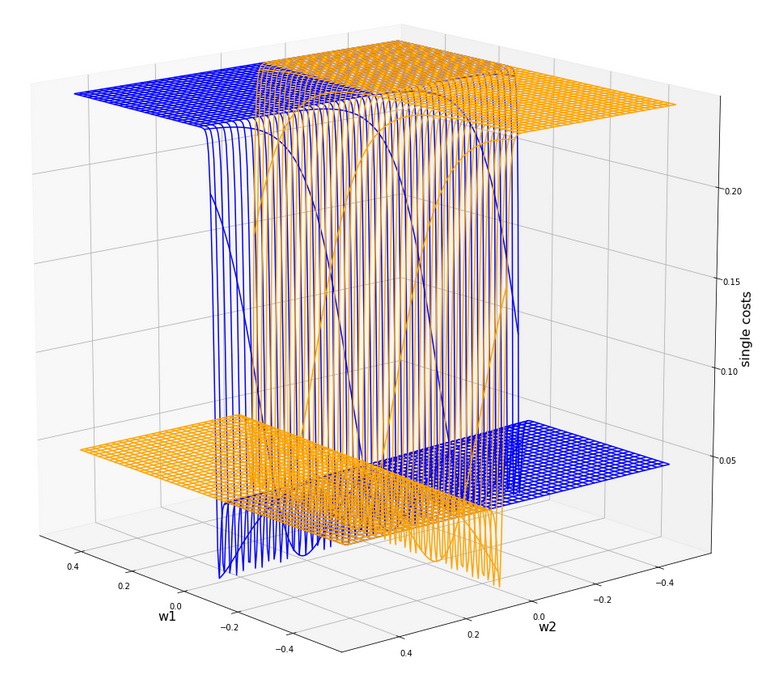Actually the cost functions for the different samples do show some systematic, though small differences. Try it out yourself … Here is the plot for samples 1,5,9 (counted from 0!).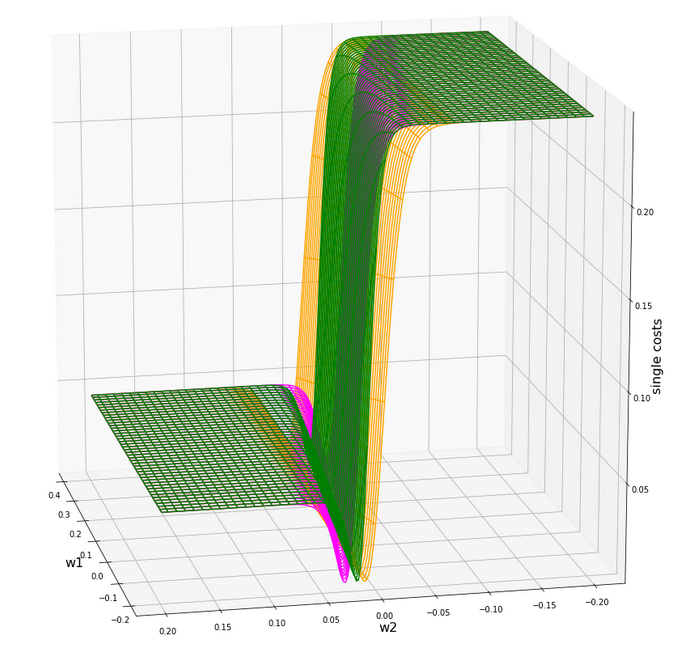You see different orientation angles in the (w1, w2)-plane?

# Total cost landscape without normalization

Now let us look at the total costs; to arrive at a comparable level with the other plots I divided the sum by 14: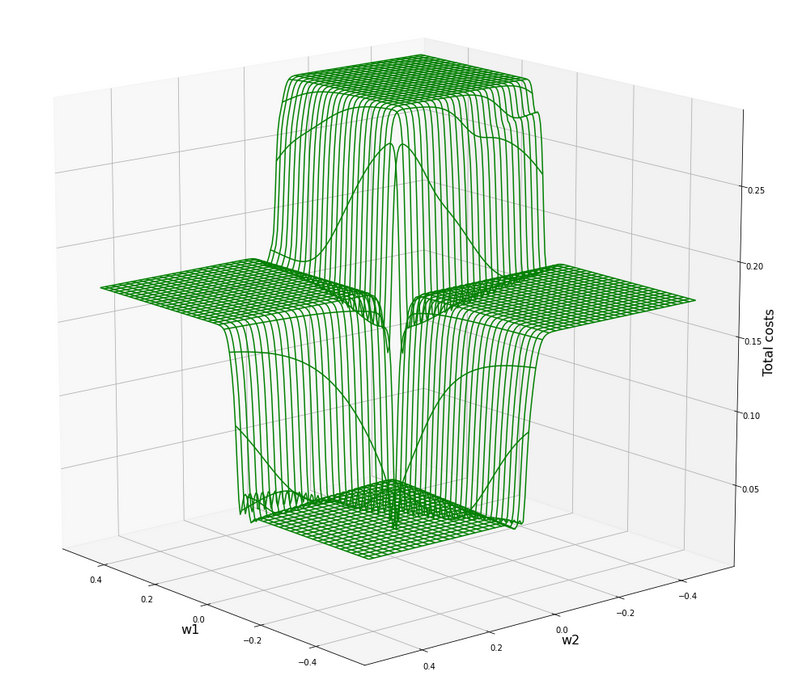All of the above cost plots look like big trouble for both the „stochastic gradient descent“ and the „batch gradient descent“ methods for „Machine Learning“ [ML]:

We clearly see the effect of the sigmoid saturation. We get almost flat areas beyond certain relatively small w1- and w2-values (|w1| > 0.02, |w2| > 0.02). The gradients in this areas will be very, very close to zero. So, if we have a starting point as e.g. (w1=0.3, w2=0.2) our gradient descent would get stuck. Due to the big input values of at least one feature.

In the center of the {w1, w2}-plane, however, we detect a steep slope to a global minimum.

But how to get there? Let us say, we start with w1=0.04, w2=0.04. The learning-rate „η“ is used to correct the weight values by

w1 = w1 – &
eta;

In the beginning you would need a big „η“ to get closer to the center due to small gradient values. However, if you choose it too big you may pass the tiny area of the minimum and just hop to an area of a different cost level with again a gradient close to zero. But you cannot decrease the learning rate fast as a remedy, either, to avoid getting stuck again.

# A view at the center of the loss function hyperplane

Let us take a closer look at the center of our disastrous total cost function. We can get there by reducing our mesh to a region defined by „-0.05 < w1, w2 < 0.05“. We get :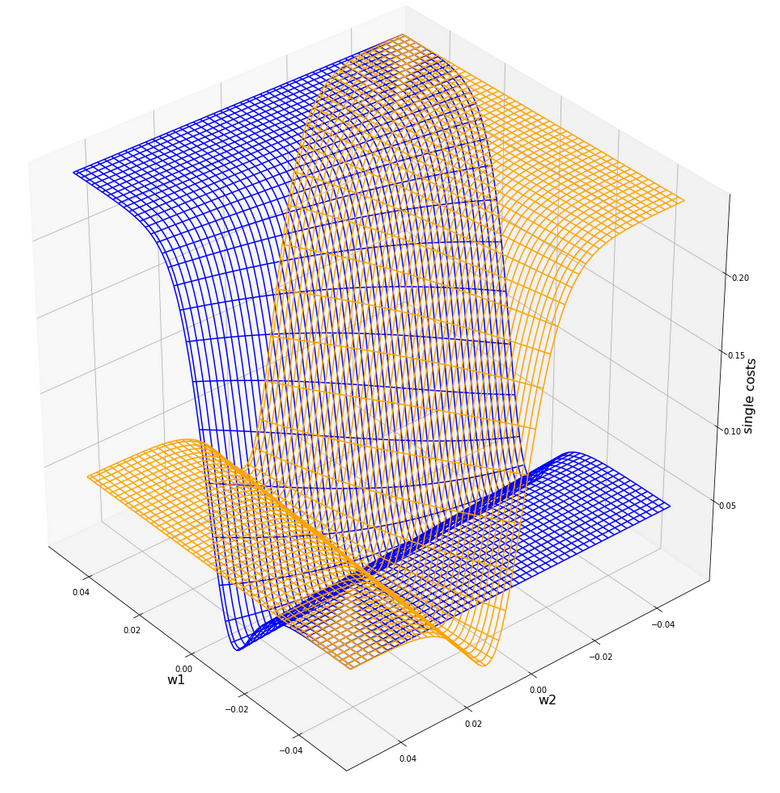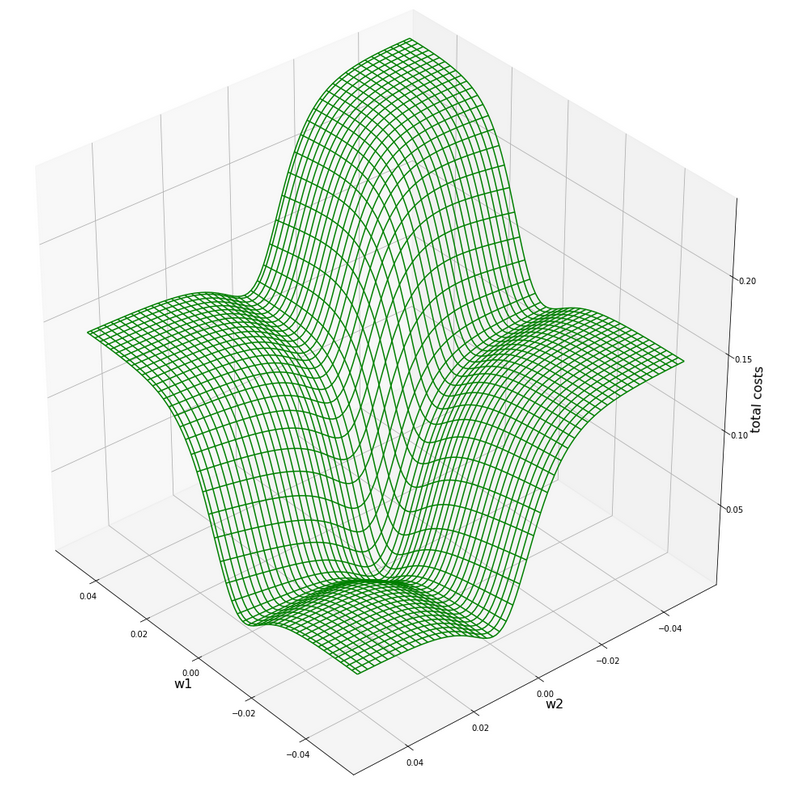This looks actually much better – on such a surface we could probably work with gradient descent. There is a clear minimum visible – and on this scale of the (w1, w2)-values we also recognize reasonably paths into this minimum. An analysis of the meshdata to get values for the minimum is possible by the following statements:

```print("min =", C.min())
pt_min = np.argwhere(C==C.min())
w1=W1[pt_min][pt_min]
w2=W2[pt_min][pt_min]
print("w1 = ", w1)
print("w2 = ", w2)
```

The result is:

min = 0.0006446277000906343
w1 = -0.004999999999999963
w2 = 0.005000000000000046

But to approach this minimum by a smooth gradient descent we would have had to know in advance at what tiny values of (w1, w2) to start with gradient descent – and choose our η suitably small in addition. This is easy in our most simplistic one neuron case, but you almost never can fulfill the first condition when dealing with real artificial neural networks for complex scenarios.

And a naive gradient descent with a standard choice of a (w1, w2)-starting point would have lead us to nowhere in our one-neuron case – as we shall see in a minute ..

Let us keep one question in mind for a while: Is there a chance that we could get the hyperplane surface to look similar to the one at the center – but for much bigger weight values?

# Some Python code for gradient descent for our one neuron scenario

Here are some useful functions, which we shall use later on to perform a gradient descent:

```
# ****************************************
# Functions for stochastic GRADIENT DESCENT
# *****************************************
import random
import pandas as pd

# derivative of expit
def d_expit(z):
exz = expit(z)
dex = exz * (1.0 - exz)
return dex

# single costs for stochastic descent
# ************************************
def dcost_sgl(w1, w2, idx, li_K1, li_K2, li_a_tgt):
z_in  = w1 * li_K1[idx] + w2 * li_K2[idx]
a_tgt = li_a_tgt[idx]
c = 0.5 * ( a_tgt - expit(z_in))**2
return c

# *********
def grad_sgl(w1, w2, idx, li_K1, li_K2, li_a_tgt):
z_in  = w1 * li_K1[idx] + w2 * li_K2[idx]
a_tgt = li_a_tgt[idx]
gradw1 = 0.5 * 2.0 * (a_tgt - expit(z_in)) * (-d_expit(z_in)) * li_K1[idx]
gradw2 = 0.5 * 2.0 * (a_tgt - expit(z_in)) * (-d_expit(
z_in)) * li_K2[idx]

def grad_tot(num_samples, w1, w2, li_K1, li_K2, li_a_tgt):
rg_idx = range(num_samples)
for idx in rg_idx:
z_in  = w1 * li_K1[idx] + w2 * li_K2[idx]
a_tgt = li_a_tgt[idx]
gradw1_idx = 0.5 * 2.0 * (a_tgt - expit(z_in)) * (-d_expit(z_in)) * li_K1[idx]
gradw2_idx = 0.5 * 2.0 * (a_tgt - expit(z_in)) * (-d_expit(z_in)) * li_K2[idx]

# total costs at given point
# ************************************
def dcost_tot(num_samples, w1, w2,li_K1, li_K2, li_a_tgt):
c_tot  = 0
rg_idx = range(num_samples)
for idx in rg_idx:
#z_in  = w1 * li_K1[idx] + w2 * li_K2[idx]
a_tgt = li_a_tgt[idx]
c_idx = dcost_sgl(w1, w2, idx, li_K1, li_K2, li_a_tgt)
c_tot += c_idx
ctot = 1.0/num_samples * c_tot
return c_tot

# Prediction function
# ********************
def predict_batch(num_samples, w1, w2,ay_k_1, ay_k_2, li_a_tgt):
shape_res = (num_samples, 5)
ResData = np.zeros(shape_res)
rg_idx = range(num_samples)
err = 0.0
for idx in rg_idx:
z_in  = w1 * ay_k_1[idx] + w2 * ay_k_2[idx]
a_out = expit(z_in)
a_tgt = li_a_tgt[idx]
err_idx = np.absolute(a_out - a_tgt) / a_tgt
err += err_idx
ResData[idx] = ay_k_1[idx]
ResData[idx] = ay_k_2[idx]
ResData[idx] = a_tgt
ResData[idx] = a_out
ResData[idx] = err_idx
err /= float(num_samples)
return err, ResData

def predict_sgl(k1, k2, w1, w2):
z_in  = w1 * k1 + w2 * k2
a_out = expit(z_in)
return a_out

def create_df(ResData):
''' ResData: Array with result values K1, K2, Tgt, A, rel.err
'''
cols=["K1", "K2", "Tgt", "Res", "Err"]
df = pd.DataFrame(ResData, columns=cols)
return df

```

With these functions a quick and dirty „gradient descent“ can be achieved by the following code:

```
# **********************************
# Quick and dirty Gradient Descent
# **********************************
b_scale_2 = False
if b_scale_2:
ay_k_1 = ay_K1
ay_k_2 = ay_K2
else:
ay_k_1 = li_K1
ay_k_2 = li_K2

li_w1_st = []
li_w2_st = []
li_c_sgl_st = []
li_c_tot_st = []

li_w1_ba = []
li_w2_ba = []
li_c_sgl_ba = []
li_c_tot_ba = []

idxc = 2

# Starting point
#***************
w1_start = -0.04
w2_start = -0.0455
#w1_start = 0.5
#w2_start = -0.5

# learn rate
# **********
eta = 0.0001
decrease_rate = 0.000000001
num_steps = 2500

# norm = 1
#eta = 0.75
#decrease_rate = 0.000000001
#num_steps = 100

# *********************
rg_j = range(num_steps)
rg_i = range(num_samples)
w1d_st = w1_start
w2d_st = w2_start
w1d_ba = w1_start
w2d_ba = w2_start

for j in rg_j:
eta = eta / (1.0 + float(j) * decrease_rate)
# loop over samples and individ. corrs
ns = num_samples
rg = range(ns)
rg_idx = random.sample(rg, num_samples)
#print("\n")
for idx in rg_idx:
#print("idx = ", idx)
li_w1_st.append(w1d_st)
li_w2_st.append(w2d_st)

# costs for special sample
cd_sgl_st = dcost_sgl(w1d_st, w2d_st, idx, ay_k_1, ay_k_2, li_a_tgt)
li_c_sgl_st.append(cd_sgl_st)

# total costs for special sample
cd_tot_st = dcost_tot(num_samples, w1d_st, w2d_st, ay_k_1, ay_k_2, li_a_tgt)
li_c_tot_st.append(cd_tot_st)
#print("j:", j, " li_c_tot[j] = ", li_c_tot[j] )

# work with total costs and total gradient
li_w1_ba.append(w1d_ba)
li_w2_ba.append(w2d_ba)
co_ba = dcost_tot(num_samples, w1d_ba, w2d_ba, ay_k_1, ay_k_2, li_a_tgt)
li_c_tot_ba.append(co_ba)

# Printed Output
# ***************
num_end = len(li_w1_st)
err_sgl, ResData_sgl = predict_batch(num_samples, li_w1_st[num_end-1], li_w2_st[num_end-1], ay_k_1, ay_k_2, li_a_tgt)
err_ba,  ResData_ba = predict_batch(num_samples, li_w1_ba[num_steps-1], li_w2_ba[num_steps-1], ay_k_1, ay_k_2, li_a_tgt)
df_sgl = create_df(ResData_sgl)
df_ba  = create_df(ResData_ba)
print("\n", df_sgl)
print("\n", df_ba)
print("\nTotal error stoch descent: ", err_sgl )
print("Total error batch descent: ", err_ba )

# Styled Pandas Output
# *******************
df_ba

```

Those readers who followed my series on a Multilayer Perceptron should have no difficulties to understand the code: I used two methods in parallel – one for a „stochastic descent“ and one for a „batch descent„:

• During „stochastic descent“ we correct the weights by a stepwise application of the cost-gradients of single samples. (We shuffle the order of the samples statistically during epochs to avoid cyclic effects.) This is done for all samples during an epoch.
• During batch gradient we apply the gradient of the total costs of all samples once during each epoch.

And here is also some code to perform some plotting after training runs:

```
# Plots for Single neuron Gradient Descent
# ****************************************
#sizing
fig_size = plt.rcParams["figure.figsize"]
fig_size = 14
fig_size = 5

fig1 = plt.figure(1)
fig2 = plt.figure(2)
fig3 = plt.figure(3)
fig4 = plt.figure(4)

ax1_1.plot(range(len(li_c_sgl_st)), li_c_sgl_st)
#ax1_1.set_xlim (0, num_tot+5)
#ax1_1.set_ylim (0, 0.4)
ax1_1.set_xlabel("epochs * batches (" + str(num_steps) + " * " + str(num_samples) + " )")
ax1_1.set_ylabel("sgl costs")

ax1_2.plot(range(len(li_c_tot_st)), li_c_tot_st)
#ax1_2.set_xlim (0, num_tot+5)
#ax1_2.set_ylim (0, y_max_err)
ax1_2.set_xlabel("epochs * batches (" + str(num_steps) + " * " + str(num_samples) + " )")
ax1_2.set_ylabel("total costs ")

ax2_1.plot(range(len(li_w1_st)), li_w1_st)
#ax1_1.set_xlim (0, num_tot+5)
#ax1_1.set_ylim (0, y_max_costs)
ax2_1.set_xlabel("epochs * batches (" + str(num_steps) + " * " + str(num_samples) + " )")
ax2_1.set_ylabel("w1")

ax2_2.plot(range(len(li_w2_st)), li_w2_st)
#ax1_2.set_xlim (0, num_to_t+5)
#ax1_2.set_ylim (0, y_max_err)
ax2_2.set_xlabel("epochs * batches (" + str(num_steps) + " * " + str(num_samples) + " )")
ax2_2.set_ylabel("w2")

ax3_1.plot(range(len(li_c_tot_ba)), li_c_tot_ba)
#ax3_1.set_xlim (0, num_tot+5)
#ax3_1.set_ylim (0, 0.4)
ax3_1.set_xlabel("epochs (" + str(
num_steps) + " )")
ax3_1.set_ylabel("batch costs")

ax4_1.plot(range(len(li_w1_ba)), li_w1_ba)
#ax4_1.set_xlim (0, num_tot+5)
#ax4_1.set_ylim (0, y_max_costs)
ax4_1.set_xlabel("epochs (" + str(num_steps) + " )")
ax4_1.set_ylabel("w1")

ax4_2.plot(range(len(li_w2_ba)), li_w2_ba)
#ax4_2.set_xlim (0, num_to_t+5)
#ax4_2.set_ylim (0, y_max_err)
ax4_2.set_xlabel("epochs (" + str(num_steps) + " )")
ax4_2.set_ylabel("w2")

```

You can put these codes into suitable cells of a Jupyter environment and start doing experiments on your PC.

# Frustration WITHOUT data normalization …

Let us get the worst behind us:
Let us use un-normalized input data, set a standard starting point for the weights and try a gradient descent with 2500 epochs.
Well, what are standard initial weight values? We can follow LeCun’s advice on bigger networks: a uniform distribution between – sqrt(1/2) and +srt(1/2) = 0.7 should be helpful. Well, we take such values. The parameters of our trial run are:

w1_start = -0.1, w2_start = 0.1, eta = 0.01, decrease_rate = 0.000000001, num_steps = 12500

You, unfortunately, get nowhere:

```        K1     K2  Tgt           Res       Err
0   200.0   14.0  0.3  3.124346e-15  1.000000
1     1.0  107.0  0.7  9.999996e-01  0.428571
2   160.0   10.0  0.3  2.104822e-12  1.000000
3    11.0  193.0  0.7  1.000000e+00  0.428571
4   220.0   32.0  0.3  1.117954e-15  1.000000
5    11.0  178.0  0.7  1.000000e+00  0.428571
6   120.0    2.0  0.3  8.122661e-10  1.000000
7    14.0  210.0  0.7  1.000000e+00  0.428571
8   195.0   12.0  0.3  5.722374e-15  1.000000
9    15.0  134.0  0.7  9.999999e-01  0.428571
10  130.0    7.0  0.3  2.783284e-10  1.000000
11    5.0  167.0  0.7  1.000000e+00  0.428571
12  185.0   10.0  0.3  2.536279e-14  1.000000
13   16.0  229.0  0.7  1.000000e+00  0.428571

K1     K2  Tgt           Res       Err
0   200.0   14.0  0.3  7.567897e-24  1.000000
1     1.0  107.0  0.7  1.000000e+00  0.428571
2   160.0   10.0  0.3  1.485593e-19  1.000000
3    11.0  193.0  0.7  1.000000e+00  0.428571
4   220.0   32.0  0.3  1.411189e-21  1.000000
5    11.0  178.0  0.7  1.000000e+00  0.428571
6   120.0    2.0  0.3  2.293804e-16  1.000000
7    14.0  210.0  0.7  1.000000e+00  0.428571
8   195.0   12.0  0.3  1.003437e-23  1.000000
9    15.0  134.0  0.7  1.000000e+00  0.428571
10  130.0    7.0  0.3  2.463730e-16  1.000000
11    5.0  167.0  0.7  1.000000e+00  0.428571
12  185.0   10.0  0.3  6.290055e-23  1.000000
13   16.0  229.0  0.7  1.000000e+00  0.428571

Total error stoch descent:  0.7142856616691734
Total error batch descent:  0.7142857142857143
```

A parameter setting like

w1_start = -0.1, w2_start = 0.1, eta = 0.0001, decrease_rate = 0.000000001, num_steps = 25000

does not bring us any further:

```        K1     K2  Tgt           Res       Err
0   200.0   14.0  0.3  9.837323e-09  1.000000
1     1.0  107.0  0.7  9.999663e-01  0.428523
2   160.0   10.0  0.3  3.496673e-07  0.999999
3    11.0  193.0  0.7  1.000000e+00  0.428571
4   220.0   32.0  0.3  7.812207e-09  1.000000
5    11.0  178.0  0.7  9.999999e-01  0.428571
6   120.0    2.0  0.3  8.425742e-06  0.999972
7    14.0  210.0  0.7  1.000000e+00  0.428571
8   195.0   12.0  0.3  1.328667e-08  1.000000
9    15.0  134.0  0.7  9.999902e-01  0.428557
10  130.0    7.0  0.3  5.090220e-06  0.999983
11    5.0  167.0  0.7  9.999999e-01  0.428571
12  185.0   10.0  0.3  2.943780e-08  1.000000
13   16.0  229.0  0.7  1.000000e+00  0.428571

K1     K2  Tgt           Res       Err
0   200.0   14.0  0.3  9.837323e-09  1.000000
1     1.0  107.0  0.7  9.999663e-01  0.428523
2   160.0   10.0  0.3  3.496672e-07  0.
999999
3    11.0  193.0  0.7  1.000000e+00  0.428571
4   220.0   32.0  0.3  7.812208e-09  1.000000
5    11.0  178.0  0.7  9.999999e-01  0.428571
6   120.0    2.0  0.3  8.425741e-06  0.999972
7    14.0  210.0  0.7  1.000000e+00  0.428571
8   195.0   12.0  0.3  1.328667e-08  1.000000
9    15.0  134.0  0.7  9.999902e-01  0.428557
10  130.0    7.0  0.3  5.090220e-06  0.999983
11    5.0  167.0  0.7  9.999999e-01  0.428571
12  185.0   10.0  0.3  2.943780e-08  1.000000
13   16.0  229.0  0.7  1.000000e+00  0.428571

Total error stoch descent:  0.7142779420120247
Total error batch descent:  0.7142779420164836
```

However:
For the following parameters we do get something:

w1_start = -0.1, w2_start = 0.1, eta = 0.001, decrease_rate = 0.000000001, num_steps = 25000

```        K1     K2  Tgt       Res       Err
0   200.0   14.0  0.3  0.298207  0.005976
1     1.0  107.0  0.7  0.603422  0.137969
2   160.0   10.0  0.3  0.334158  0.113860
3    11.0  193.0  0.7  0.671549  0.040644
4   220.0   32.0  0.3  0.294089  0.019705
5    11.0  178.0  0.7  0.658298  0.059574
6   120.0    2.0  0.3  0.368446  0.228154
7    14.0  210.0  0.7  0.683292  0.023869
8   195.0   12.0  0.3  0.301325  0.004417
9    15.0  134.0  0.7  0.613729  0.123244
10  130.0    7.0  0.3  0.362477  0.208256
11    5.0  167.0  0.7  0.654627  0.064819
12  185.0   10.0  0.3  0.309307  0.031025
13   16.0  229.0  0.7  0.697447  0.003647

K1     K2  Tgt       Res       Err
0   200.0   14.0  0.3  0.000012  0.999961
1     1.0  107.0  0.7  0.997210  0.424586
2   160.0   10.0  0.3  0.000106  0.999646
3    11.0  193.0  0.7  0.999957  0.428510
4   220.0   32.0  0.3  0.000009  0.999968
5    11.0  178.0  0.7  0.999900  0.428429
6   120.0    2.0  0.3  0.000771  0.997429
7    14.0  210.0  0.7  0.999980  0.428543
8   195.0   12.0  0.3  0.000014  0.999953
9    15.0  134.0  0.7  0.998541  0.426487
10  130.0    7.0  0.3  0.000555  0.998150
11    5.0  167.0  0.7  0.999872  0.428389
12  185.0   10.0  0.3  0.000023  0.999922
13   16.0  229.0  0.7  0.999992  0.428560

Total error single:  0.07608269490258893
Total error batch:  0.7134665897677123
```

By pure chance we found a combination of starting point and learning-rate for which we – by hopping around on the flat cost areas – we accidentally arrived at the slope area of one sample and started a gradient descent. This did however not (yet) happen for the total costs.
We get a minimum around (w1=-0.005,w2=0.005) but with a big spread of 0.0025 for each of the weight values.

# Intermediate Conclusion

We looked at a simple perceptron scenario with one computing neuron. Our solitary neuron should learn to distinguish between input data of two distinct and separate data clusters in a 2-dimensional feature space. The feature data change between big and small values for different samples. The neuron used the sigmoid-function as activation and output function. The cost function for all samples shows a minimum at a tiny area in the weight space. We found this minimum with the help of a fine grained and mesh-based analysis of the cost values. However, such an analysis is not applicable to general ML-scenarios.

The problem we face is that due to the saturation properties of the sigmoid function the minimum cannot be detected automatically via gradient descent without knowing already precise details about the solution. Gradient descent does not work – we either get stuck on areas of nearly constant costs or we hop around between different plateaus of the cost function – missing a tiny location in the (w1, w2)-parameter space for a real descent into the existing minimum.

We need to find a way out of this dilemma. In the next article

A single neuron perceptron with sigmoid activation function – II – normalization to overcome saturation

I shall show that normalization opens such a way.